# HSSlive: Plus One & Plus Two Notes & Solutions for Kerala State Board

## इयत्ता दहावी विज्ञान धडा लेन्स मराठी स्वाध्याय PDFइयत्ता दहावी विज्ञान धडा लेन्स मराठी स्वाध्याय PDF

या लेखात, आम्ही विज्ञान धडा लेन्स विषयासाठी इयत्ता दहावी मराठी सोल्यूशन्स देऊ. इयत्ता दहावी मधील विद्यार्थी पाठ्यपुस्तकांमध्ये उपस्थित असलेल्या व्यायामांसाठी प्रश्न आणि उत्तरे डाउनलोड आणि कॉपी करण्यास सक्षम असतील.

इयत्ता दहावी विज्ञान धडा लेन्साच्या पुस्तकात महाराष्ट्र बोर्डाच्या अभ्यासक्रमातील सर्व प्रश्नांचा समावेश आहे. येथे सर्व प्रश्न पूर्ण स्पष्टीकरणासह सोडवले आहेत आणि डाउनलोड करण्यासाठी विनामूल्य उपलब्ध आहेत. महाराष्ट्र बोर्ड इयत्ता दहावी विज्ञान धडा लेन्साचे पुस्तक खाली दिले आहे. आम्‍हाला आशा आहे की आमच्‍या इयत्‍ता दहावी वीच्‍या विज्ञान धडा लेन्साचे पुस्‍तक तुमच्‍या अभ्यासात मदत करेल! जर तुम्हाला आमचे इयत्ता दहावी चे पुस्तक आवडले असेल तर कृपया ही पोस्ट शेअर करा.

## इयत्ता दहावी विज्ञान धडा लेन्स स्वाध्याय

 मंडळाचे नाव Maharashtra Board ग्रेडचे नाव दहावी विषय विज्ञान धडा लेन्स वर्ष 2022 स्वरूप PDF/DOC प्रदाता hsslive.co.in अधिकृत संकेतस्थळ mahahsscboard.in

## समाधानासह महाराष्ट्र बोर्ड आठवा स्वाध्याय कसे डाउनलोड करायचे?

महाराष्ट्र बोर्ड दहावी स्वाध्याय PDF डाउनलोड करण्यासाठी खालील स्टेप्स फॉलो करा:

1. वेबसाइट- Hsslive ला भेट द्या. 'स्वाध्याय' लिंकवर क्लिक करा.
2. महा बोर्ड दहावी स्वाध्याय PDF पहा.
3. आता महाराष्ट्र बोर्ड दहावी स्वाध्याय तपासा.
4. डाउनलोड करा आणि भविष्यातील संदर्भांसाठी जतन करा.

## इयत्ता दहावी विज्ञान धडा लेन्स स्वाध्याय उपाय

इयत्ता दहावी स्वाध्याय मधील विद्यार्थी खालील लिंक्सवरून विज्ञान धडा लेन्साचे उपाय डाउनलोड करू शकतील.

Question 1.
Match the columns in the following table and explain them:

 Column 1 Column 2 Column 3 Farsightedness Nearby object can be seen clearly Bifocal  lens Presbyopia Faraway object can be seen clearly Concave  lens Nearsightedness Problem of old age Convex  lens

 Column 1 Column 2 Column 3 Farsightedness Faraway object can be seen clearly Convex  lens Presbyopia Problem of old age Bifocal  lens Nearsightedness Nearby object can be seen clearly Concave  lens

1. Farsightedness:
Hypermetropia or farsightedness is the defect of vision in which a human eye can see distant objects clearly but is unable to see nearby objects clearly.
In this case the image of a nearby object would fall behind the retina instead of on the retina.

Possible reasons for hypermetropia:
(1) Curvature of the cornea and the eye lens decreases. Hence, the converging power of the eye lens becomes less.
(2) The distance between the eye lens and retina decreases (relative to the normal eye) and the focal length of the eye lens becomes very large due to the flattening of the eyeball.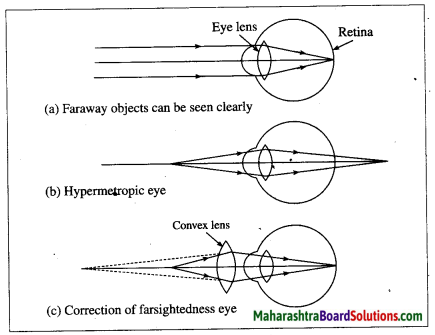2. Presbyopia:
Presbyopia is the defect of vision in which aged people find it difficult to see nearby objects
comfortably and clearly without spectacles.

Reason of presbyopia: The power of accommodation of eye usually decreases with ageing. The muscles near the eye lens lose their ability to change the focal length of the lens.

Therefore, the near point of the eye lens shifts rarther from the eye, This defect is corrected using a. convex lens of appropriate power. The lens converges light rays before they fall on the eye lens such that the action of the eye lens forms the image on the retina.

3. Nearsightedness:
Myopia or nearsightedness is the defect of vision in which a human eye can see nearby objects distinctly but is unable to see distant objects clearly as they appear indistinct.
In this case the image of a distant object is formed in front of the retina instead of on the retina.
[Figs. 7.29 (a), 7.29 (b)]

Possible reasons for myopia:
(1) The curvature of the cornea and the eye lens increases. The muscles near the lens cannot relax so that the converging power of the lens remains large. (2) The distance between the eye lens and the retina increases as the eyeball elongates.

Myopia is corrected using a suitable concave lens. Light rays are diverged by the concave lens before they strike the eye lens. A concave lens of proper focal length is chosen to produce the required divergence. Hence, after the converging action of the eye lens, the image is formed on the retina. [Fig. 7.29 (c)]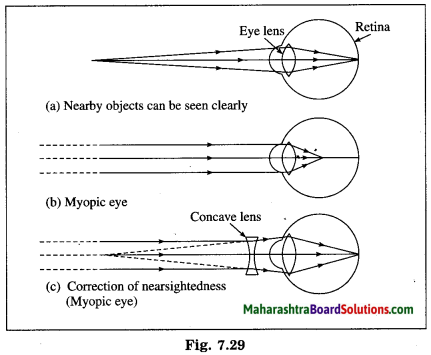Question 2.
Draw a figure explaining various terms related to a lens.
(1) Centre of curvature (C): The centres of the spheres whose parts form the surfaces of a lens are called the centres of curvature of the lens. A lens has two centres of curvature C1, and Cfor its two spherical surfaces.

(2) Radii of curvature (R1, R2): The radii of the spheres whose parts form surfaces of a lens are called the radii of curvature of the lens.

(3) Principal axis: The imaginary straight line passing through the two centres of curvature of a lens is called the principal axis of the lens.

(4) Optical centre (O): The point inside a lens on the principal axis, through which light rays pass without changing their path is called the optical centre (O) of the lens.

(5) Principal focus (F): When light rays parallel to the principal- axis are incident on a convex lens, they converge at a point on the principal axis. This point is called the principal focus (F) of the convex lens. Light rays travelling parallel to the principal axis of a concave lens diverge after refraction in such a way that they appear to be coming out of a point on the principal axis. This point is called the principal focus of the concave lens. A lens has two principal foci F1 and F2.
[Note: In this chapter, the terms focus and the principal focus are used in the same sense.]

(6) Focal length (f): The distance between the optical centre and the principal focus of a lens is called the focal length (f) of the lens.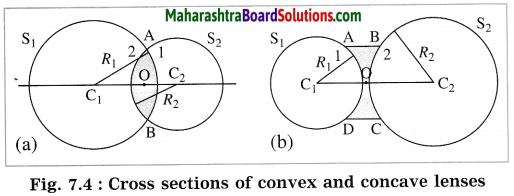C1, C2: Centres of curvature, R1, R2: Radii of curvature, O: Optical centre.
The cross sections of convex and concave lenses are shown in parts (a) and (b) of Fig. 7.4. The surface marked as 1 is part of sphere S1 while the surface marked as 2 is part of sphere S2.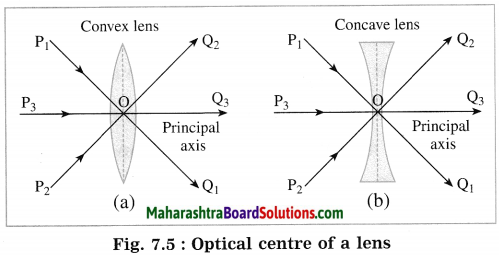P1, P2, P3: Incident rays of light,
Q1, Q2, Q3: Refracted rays of light, O: Optical centre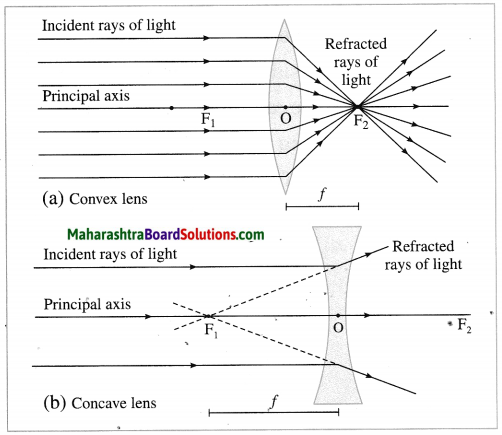F1, F2: Principal foci of the lens, f: Focal length of the lens

Question 3.
At which position will you keep an object in front of a convex lens so as to get a real image of the same size as the object? Draw a figure.
At 2F1.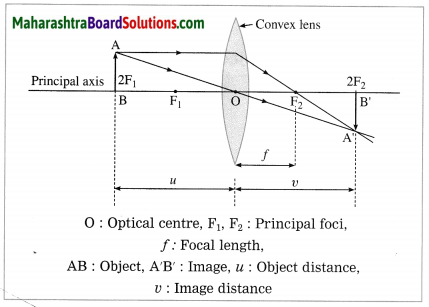Question 4.
Give scientific reasons:
a. A simple microscope is used for watch repairs.
(1) when an object is placed within the focal length of a magnifying glass or simple microscope (convex lens), its larger and erect image is obtained on the same side of the lens as that of the object.

(2) By adjusting the distance between the object and the lens, the image can be obtained at the minimum distance of distinct vision. Thus, a watch repairer can see the minute parts of a watch more clearly with the aid of a magnifying glass (a simple microscope) than with the naked eye, without any stress on the eye. Hence, watch repairers use a magnifying glass (a simple microscope) while repairing the watches.

b. One can sense colours only in bright light.
(1) The retina in the eye is made of many light sensitive cells. The rod-shaped cells respond to the intensity of light while the cone-shaped cells j respond to various colours.
(2) The cone-shaped cells do not respond to faint light. They function only in bright light. Hence, one can sense colours only in bright, light.

c. We cannot clearly see an object kept at a distance less than 25 cm from the eye.
(1) When we try to see a nearby object, the eye lens becomes more rounded and its focal length decreases. Then a clear image of the object is formed on the retina of the eye.
(2) The focal length of the eye lens cannot be decreased beyond some limit. Therefore we cannot clearly see an object kept at a distance less than 25 cm from the eye.

Question 5.
Explain the working of an astronomical telescope using refraction of light.
Construction of a refracting telescope: It consists of two convex lenses called the objective lens (directed towards the object) and the eyepiece (directed towards the eye). The focal length and diameter of the objective lens are respectively greater than the focal length and diameter of the eyepiece. The objective lens is fitted at one end of a long metal tube.

A metal tube of smaller diameter is fitted in this metal tube and the eyepiece is fitted at the outer end of the smaller tube. With the help of a screw it is possible to change the distance between the eyepiece and the objective lens by sliding the tube fitted with the eyepiece. The principal axes of the objective lens and the eyepiece are along the same line. A telescope is usually mounted on a stand.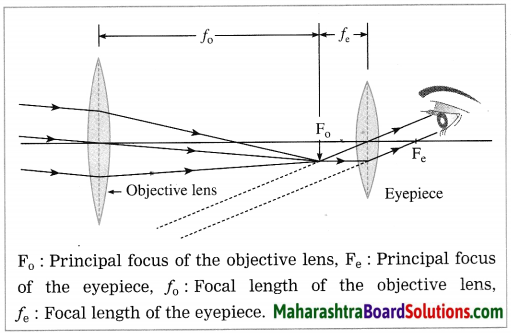Working: When the objective lens is pointed towards the distant object to be observed, the rays of light from the distant object, which are almost parallel to each other, pass through the objective lens. The objective lens collects maximum amount of light as it is large in size. It forms a real, inverted and diminished image in the focal plane of the objective lens. Now, the position of the eyepiece is adjusted such that this image falls just within the focal length of the eyepiece and serves as the object for the eyepiece which works as a simple microscope.

The final image is highly magnified, virtual, on the same side as that of the object and inverted with respect to the original object. The final image can be observed by keeping the eye close to the eyepiece. If the image formed by the objective lens lies in the focal plane of the eyepiece, the final image is formed at infinity.

Question 6.
Distinguish between the following:
a. Farsightedness (Hypermetropia) and Nearsightedness (Myopia).
Farsightedness:

1. In hypermetropia, a human eye can see distant distinctly but is unable to see nearby objects clearly.
2. In this case, the image of a nearby object would be formed behind the retina.
3. This defect can be corrected using a convex lens of appropriate power.

Nearsightedness:

1. In myopia, a human eye can see near objects distinctly, but is unable to see distant objects clearly.
2. In this case, the image of a distant object is formed in front of the retina.
3. This defect can be corrected using a concave lens of appropriate power.

b. Concave lens and Convex lens.
Concave lens:

1. A concave lens has its surfaces curved inwards.
2. It is thicker at the edges than in the middle.
3. It can form only a virtual image.
4. It can form only a diminished image.

Convex lens:

1. A convex lens has its surfaces puffed up outwards.
2. It is thicker in the middle than at the edges.
3. It can form a real image as well as a % virtual image.
4. It can form a magnified, diminished or the same sized image (relative to the object) depending on the position of the object.

Question 7.
What is the function of the iris and the muscles connected to the lens in the human eye?
When the incident light is very bright, the muscles of the iris stretch to reduce the size of the pupil. When the incident light is dim, the muscles of the iris relax to increase the size of the pupil. Thus, the iris controls the size of the pupil and thereby regulates the amount of light entering the eye. (Fig. 7.26)

When a distant object is to be observed, the ciliary muscles relax so that the eye lens becomes flat. This increases the focal length of the lens. Therefore, a sharp image of the distant object is formed on the retina.
Thus, we can see a distant object clearly. (Fig. 7.27)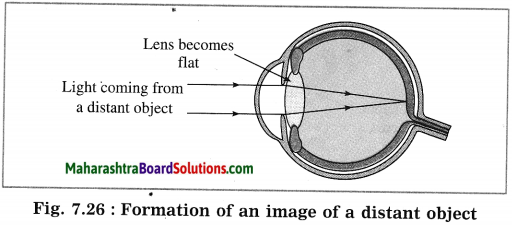when an object closer to the eye is to be observed. the ciliary muscles contract increasing the curvature. of the eye lens. The eye lens, therefore, becomes rounded. This decreases the focal length of the lens. Therefore, a sharp image of the nearby object is formed on the retina. Thus, we can see a nearby object clearly. (Fig. 7.27)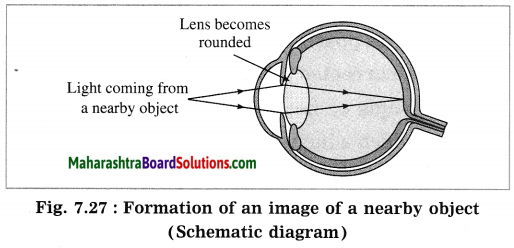Question 8.
Solve the following examples:
i. Doctor has prescribed a lens having I power + 1.5 D. What will be the focal length of the lens? What is the type of the lens and what must be the defect of vision?
Solution:
Data: P = + 1.5 D, f = ?
Focal length of the lens, f = 1𝑃=11.5D
1015 m = 0.6667 m = 0.67 m
P is positive. This shows that the lens is convex. The defect of vision is farsightedness (hypermetropia).

ii. 5 cm high object is placed at a distance of 25 cm from a converging lens of focal length of 10 cm. Determine the position, size and type of the image.
Solution:
Data: Converging lens, f = 10 cm
u = – 25 cm, h1 = 5 cm, v = ?, h2 = ?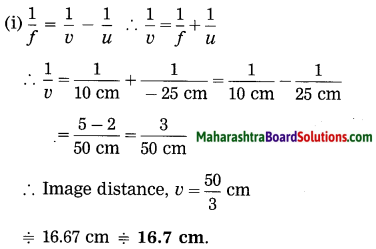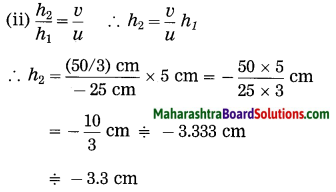The height of the image = -3.3 cm (inverted image ∴ minus sign).
(iii) The image is real, inverted and smaller than the object.

iii. Three lenses having powers 2, 2.5 and 1.7 D are kept touching in a row. What is the total power of the lens combination?
Solution:
Data: P1 = 2 D, P2 = 2.5 D, P3 = 1.7 D, P = ?
Total power of the lens combination,
P = P1 +P2 + P3
= 2 D + 2.5 D + 1.7 D
= 6.2 D.

iv. An object kept 60 cm from a lens gives a virtual image 20 cm in front of the lens. What is the focal length of the lens? Is it a converging lens or diverging lens?
Solution:
Data: u = -60 cm, v = -20 cm, f = ?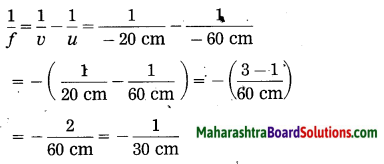∴ The focal length of the lens, f = – 30 cm. As f is negative, it is a diverging lens.

Project:

Question 1.
Make a Powerpoint presentation about the construction and use of binoculars. (Do it your self)

Can you recall? (Text Book Page No. 80)

Question 1.
Indicate the following terms related to spherical mirrors in figure 7.1: pole, centre of curvature, radius of curvature, principal focus.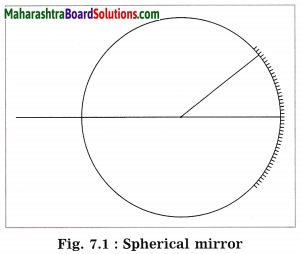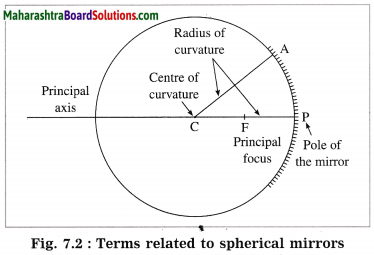Question 2.
How are concave and convex mirrors constructed?
The given part of a hollow spherical glass can be converted into a concave mirror by (i) polishing (silvering) its inner side (inner surface or concave surface) to make it reflecting or (ii) coating its outer side with a thin layer of silver and painting it with red colour to protect the silver coating.
[Note: Case (i) corresponds to the front surface silvered concave mirror.]

The given part of a hollow spherical glass can be converted into a convex mirror by (i) polishing (silvering) its outer side (outer surface or convex surface) to make it reflecting or (ii) coating its inner side with a thin layer of silver and painting it with red colour to protect the silver coating.
[Note: Case (i) corresponds to the front surface silvered convex mirror. ]

Use your brain power! (Text Book Page No. 85)

Question 1.
From equations (1) and (2) what is the relation between h1, h2, u and v?
M = 21 …….(1)
Also, M = 𝑣( image distance )𝑢 (object distance) ……(2)
21 = 𝑣𝑢

Try this (Text Book Page No. 88)

(1) Try to read a book keeping it very far from your eyes.
(2) Try to read a book keeping it very close to your eyes.
(3) Try to read a book keeping it at a distance of 25 cm from your eyes.
At which time do you see the alphabets clearly? Why?
Minimum distance of distinct vision: Though the focal length of the eye lens is adjustable, it cannot be decreased below a certain limit. Hence, if an object is very close to the eye, it cannot be seen clearly. For a normal human eye, the minimum distance from the eye at which an object is clearly visible without stress on the eye, is called the minimum distance of distinct vision. For the normal human eye, it is 25 cm.

Use your brain power! (Text Book Page No. 89)

Question.
(1) Why do we have to bring a small object near the eyes in order to see it clearly?
(2) If we bring an object closer than 25 cm from the eyes, when can we not see it clearly even though it subtends a bigger angle at the eye?
(1) when a small object is brought near the eyes, its apparent size increases. Therefore, it is
seen clearly.

(2) Minimum distance of distinct vision: Though the focal length of the eye lens is adjustable, it cannot be decreased below a certain limit. Hence, if an object is very close to the eye, it cannot be seen clearly. For a normal human eye, the minimum distance from the eye at which an object is clearly visible without stress on the eye, is called the minimum distance of distinct vision. For the normal human eye, it is 25 cm.

Try this (Text Book Page No. 91)

Question 1.
Take a burning incense stick in your hand and rotate it fast along a circle.
A circle of red light is seen.

Question 2.
Draw a cage on one side of a cardboard and a bird on the other side. Hang the cardboard with the help of a thread. Twist the thread and leave It. What do you see and why?
The bird appears to be inside the cage. This happens due to persistence of vision.
Persistence of vision: We see an object when its image is formed on the retina. The image disappears when the object is removed from our sight. But this is not instantaneous and the image remains imprinted on the retina for about 116 th of a second after the removal of the object. The sensation on the retina persists for a while. This effect is known as the persistence of vision.

It is due to persistence of vision that we continue to see the object in its position for about 116 th of a second after it is removed.
Example: When a burning stick of incense is moved fast in a circle, a circle of red light is seen.

Can you tell? (Text Book Page No. 91)

Question 1.
How do we perceive different colours?
(1) In nature we field objects of various colours. Perception of colour means to be able to respond to colour.
(2) We can distinguish between various colours due to perception of colour.
(3) The cone-shaped cells on the retina of the eye respond to the various colours when light is bright and communicate to the brain about the colours of the image formed on the retina. This gives us the proper idea about the colours of the object.
(4) If, in the retina of a person, the cone-shaped cells responding to certain specific colours are absent, the person is unable to distinguish between the colours. As a result, he lacks perception of colour.

Fill in the blanks and rewrite the statements:

Question 1.
The focal length of a………..lens is positive.
The focal length of a convex lens is positive.

Question 2.
The focal length of a………..lens is negative.
The focal length of a concave lens is negative.

Question 3.
The magnification produced by a………..lens is always positive.
The magnification produced by a concave lens is always positive.

Question 4.
The power of a………..lens is positive.
The power of a convex lens is positive.

Question 5.
The power of a………..lens is negative.
The power of a concave lens is negative.

Question 6.
The focal length of a lens with power 2.5 D is………..
The focal length of a lens with power 2.5 D is 40 cm (0.4 m).

Question 7.
The power of a lens with focal length 20 cm is………..
The power of a lens with focal length 20 cm is 5D.

Question 8.
The minimum distance of distinct vision for a normal human eye is………..
The minimum distance of distinct vision for a normal human eye is 25 cm.

Question 9.
If two lenses with focal lengths 10 cm and 20 cm respectively are kept in contact with each other, the effective power of the combination is………..
If two lenses with focal lengths 10 cm and 20 cm respectively are kept in contact with each other, the effective power of the combination is 15 D.

Question 10.
A………..lens is used as a simple microscope.
A convex lens is used as a simple microscope.

Rewrite the following statements by selecting the correct options:

Question 1.
Inside water, an air bubble behaves………..
(a) like a flat plate
(b) like a concave lens
(c) like a convex lens
(d) like a concave mirror
Inside water, an air bubble behaves like a concave lens.

Question 2.
…………represents the lens formula.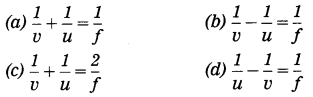(b) 1𝑣1𝑢=1𝑓 represents the lens formula.

Question 3.
The power of a convex lens of focal length 25 cm is………..
(a) +4.0 D
(b) 0.25 D
(c) -4.0 D
(d) -0.4D
The power of a convex lens of focal length 25 cm is +4.0 D

Question 4.
A lens does not produce any deviation of a ray of light passing through………..
(a) it’s centre of curvature
(b) it’s optical centre
(c) it’s principal focus
(d) an axial point at a distance 2F from its centre
A lens does not produce any deviation of a ray of light passing through its optical centre.

Question 5.
The image formed by a concave lens is always………..
(a) virtual and erect
(b) real and erect
(c) virtual and inverted
(d) real and inverted
The image formed by a concave lens is always virtual and erect.

Question 6.
A convex lens forms a virtual image of an object placed………..
(a) at infinity
(b) at a distance 2F from the lens
(c) at a distance F from the lens
(d) between the principal focus and the optical centre of the lens.
A convex lens forms a virtual image of an object placed between the principal focus and the optical centre of the lens.

Question 7.
When an object is placed at 2F1 of a convex lens, its image is formed………..
(a) at F1
(b) at 2F2
(c) beyond 2F2
(d) on the same side as the object
When an object is placed at 2F1 of a convex lens, its image is formed at 2F2

Question 8.
To obtain an image of the same size as that of an object with the help of a convex lens, the object should be placed………..
(a) at infinity
(b) beyond F1
(c) between F1 and 2F1
(d) at 2F1
To obtain an image of the same size as that of an object with the help of a convex lens, the object should be placed at 2F1

Question 9.
When an object is placed between O and F1 in front of a convex lens, the image formed is ………..
(a) enlarged and erect
(b) diminished and erect
(c) real and enlarged
(d) diminished and inverted
When an object is placed between O and F1 in front of a convex lens, the image formed is enlarged and erect.

Question 10.
When an object is placed at any finite distance from a concave lens, the image is formed ………..
(a) between F1 and 2F1
(b) beyond 2F1
(c) at F1
(d) between F1 and O on the same side as the object.
When an object is placed at any finite distance from a concave lens, the image is formed between F1 and O on the same side as the object.

Question 11.
A student obtained a clear image of window grills on the screen. But the teacher told him to get the image of a tree far away, instead of the window. To get a clear image, the lens must be ……….. (Practice Activity Sheet – 2)
(a) moved towards the screen
(b) moved away from the screen
(c) moved behind the screen
(d) moved far away from the screen
A student obtained a clear image of window grills on the screen. But the teacher told him to get the image of a tree far away, instead of the window. To get a clear image, the lens must be moved towards the screen.

Question 12.
The image obtained while finding the focal length of a convex lens is ……….. (Practice Activity Sheet – 3)
(a) real and erect
(b) virtual and erect
(c) real and inverted
(d) virtual and inverted
The image obtained while finding the focal length of a convex lens is real and inverted.

Question 13.
Yash found out f1 and f2 of a symmetric convex lens experimentally. Then which of the following conclusions is true? (Practice Activity Sheet – 4)
(a) f1 = f2
(b) f1 > f2
(c) f1 < f2
(d) f1 ≠ f2
(a) f1 = f2

State whether the following statements are true or false: (If a statement is false, correct it and rewrite it.)

Group (A)

Question 1.
Power of a lens, P = 1𝑓.
True.

Question 2.
If the power of a lens is 2 D, its focal length = 0.5 m.
True.

Question 3.
A concave lens is a converging lens. (March 2019)
False. (A concave lens is a diverging lens.)

Question 4.
A convex lens is a diverging lens.
False. (A convex lens is a converging lens.)

Question 5.
A concave lens always forms a virtual image.
True.

Question 6.
A convex lens always forms a virtual image.
False. (A convex lens forms a real image or a virtual image depending on the object distance.)

Question 7.
Due to the light sensitive cells in the eye, we get information about the brightness or dimness of the object and the colour of the object.
True.

Question 8.
The focal length of a concave lens is negative.
True.

Question 9.
The magnification produced by a concave lens is positive or negative depending on the object distance.
False. (The magnification produced by a concave lens is always positive.)

Question 10.
The magnification produced by a convex lens is positive or negative depending on the object distance.
True.

Question 11.
A concave lens is used as a magnifying glass.
False. (A convex lens is used as a magnifying glass.)

Question 12.
A convex lens is used as a simple microscope.
True.

Question 13.
A concave lens is used to correct myopia.
True.

Question 14.
A convex lens is used to correct hypermetropia.
True.

Group (B)

Question 1.
When red light falls on the eyes, the cells responding to red light get excited more than those responding to other colours and we get the sensation of red colour.
True.

Question 2.
When an object is placed in front of a concave lens, its image is obtained on the opposite side of the object.
False. (When an object is kept in front of a concave lens, its image is obtained on the same side of the lens as the object.)

Question 3.
The image formed by a concave lens is always virtual.
True.

Question 4.
The principal focus of a convex lens is virtual.
False. (The principal focus of a convex lens is real.)

Question 5.
An object of height 2 cm forms an image of height 3 cm when placed in front of a concave lens.
False. (An object of height 2 cm forms an image of height less than 2 cm when placed in front of a concave lens.)

Question 6.
Absence of rod like cells results in colour blindness.
False. (Absence of conical cells results in colour-blindness.)

Question 7.
Nearsightedness can be corrected using spectacles having convex lenses.
False. (Nearsightedness can be corrected using spectacles having concave lenses.)

Question 8.
Farsightedness can be corrected using spectacles having convex lenses of suitable focal length.
True.

Question 9.
As one grows old, ciliary muscles become weak.
True.

Question 10.
In a simple microscope, the object is placed within the focal length of the convex lens.
True.

Question 11.
A compound microscope forms an erect and real image of a small object.
False. (A compound microscope forms an inverted and virtual image of a small object.)

Question 12.
In a compound microscope, a real image acts as an object for the eyepiece.
True.

Question 13.
In television, we see a continuous picture due to persistence of vision.
True.

Question 14.
The conical cells can respond differently to red, green and blue colours.
True.

Question 15.
The rod like cells respond to colours and communicate the presence of colours in the retinal image of the brain.
False. (The rod like cells respond to the intensity of light and communicate the degree of brightness and darkness, to the brain.)

Question 16.
The conical cells respond to the intensity of light and communicate the degree of brightness and darkness to the brain.
False. (The conical cells respond to colours and communicate the presence of colours in the retinal image to the brain.)

Question 17.
Generally, using the same objective lens, but different eyepieces, different magnification can be obtained.
True.

Find the odd one out and give the reason:

Question 1.
Simple microscope, Compound microscope, Telescope, Myopia.
Myopia. It is a defect of vision; others are instruments.

Question 2.
Myopia, Presbyopia, Hypermetropia, Spectrometer.
Spectrometer. It is an instrument; others are defects of vision.

Question 3.
Presbyopia, Retina, Nearsightedness, Farsightedness.
Retina. It is a part of the eye; others are defects of vision.

Question 4.
Compound microscope, Kaleidoscope, Simple microscope, Astronomical telescope.
Kaleidoscope. Others are optical instruments.

Question 5.
TV, Motion picture, Complete circle formed by a revolving burning incense stick, Colour blindness.
Colour-blindness. Others are examples of persistence of vision.

Question 6.
Planets, Stars, Satellites, Rainbow.
Rainbow. Others are celestial bodies.

Considering the correlation between the words of the first pair, pair the third word accordingly with proper answer:

Question 1.
Nearsightedness: Elongated eyeball :: Farsightedness:………
Flattened eyeball

Question 2.
Convex lens : Converging :: Concave lens :………..
Diverging

Question 3.
Object at 2F1 of a convex lens : Image at 2F2 :: Object at F1 :………..
Image on the opposite side at infinity

Question 4.
Magnification positive : Erect image :: Magnification negative :………..
Inverted image

Question 5.
Convex lens : Positive power of the lens :: Concave lens:
Negative power of the lens.

Question 6.
1𝑓( in metre ) : Power of the lens (in dioptre) ::  Image distance  Object distance
Magnification.

Question 7.
Focal length : Metre :: Power of a lens :………..
Dioptre.

Question 8.
Iris : Pupil :: Ciliary muscles :……….
Eye lens

Question 9.
Nearsightedness : Concave lens :: Farsightedness :………..
Convex lens

Question 10.
Nearsightedness : Image in front of the retina :: Farsightedness :………..
Image behind the retina

Question 11.
Observation of stars and planets : Telescope :: Repairing a watch :……….
Simple microscope

Question 12.
Cinema : Persistence of vision :: Rainbow :………
Refraction, dispersion and internal reflection of light.

Match the following:

Question 1.

 Column A Column B (1) Conical cells (a) Intensity of light (2) Rod like cells (b) Colour of an image (3) Pupil (c) Iris (4) Cornea (d) Aperture (e) Transparent

(1) Conical cells – Colour of an image
(2) Rod like cells – Intensity of light
(3) Pupil – Aperture
(4) Cornea – Transparent.

Question 2.

 Column A Column B (1) Magnification (a) 1𝑓 (2) Power of a lens (b) ℎ2ℎ1 (3) Focal length (c) f (4) Distance of an object from a lens (d) u (e) ℎ1ℎ2

(1) Magnification: 21
(2) Power of a lens: 1𝑓
(3) Focal length: f
(4) Distance of an object from a lens: u.

Question 3.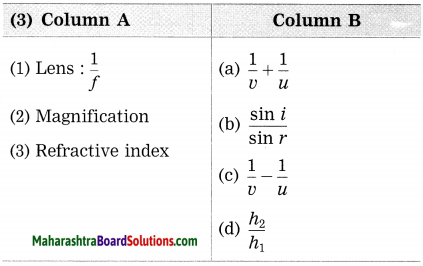(1) Lens: 1𝑓:1𝑣1𝑢
(2) Magnification: 21
(3) Refractive index: sin𝑖sin𝑟

Question 4.

 Column A Column B (Convex lens) (a) Image virtual, erect and enlarged (1) Object at 2F1 (b) Image real, inverted and of the same size (2) Object between F1 and 2F1 (c) Image real, inverted and highly diminished (3) Object between O and F1 (d) Image real, inverted and highly enlarged (4) Object at infinity (e) Image real, inverted and enlarged

(1) Object at 2F1 – Image real, inverted and of the same size
(2) Object between F1 and 2F1 – Image real, inverted and enlarged
(3) Object between O and F1 – Image virtual, erect and enlarged
(4) Object at infinity – Image real, inverted and highly diminished

Question 5.

 Column A Column B (1) Nearsightedness (a) Ciliary muscles become weak (2) Farsightedness (b) Image in front of the retina (3) Presbyopia (c) Colour-blindness (d) Image behind the retina

(1) Nearsightedness – Image in front of the retina
(2) Farsightedness – Image behind the retina
(3) Presbyopia – Ciliary muscles become weak

Question 6.

 Column A Column B (1) Convex lens (a) To see small objects clearly (2) Astronomical telescope (b) To observe minute objects (3) Compound microscope (c) To observe astronomical objects such as stars, planets, etc. (4) Simple microscope (d) Presbyopia (e) Power of a lens

(1) Convex lens – Presbyopia
(2) Astronomical telescope – To observe astronomical objects such as stars, planets, etc.
(3) Compound microscope – To observe minute objects
(4) Simple microscope – To see small objects clearly.

Question 7.

 Column A Column B (1) Persistence of vision (a) Lenses and mirrors are used (2) Reflecting telescope (b) To see objects far away from us (3) Telescope (c) Motion picture (4) Compound microscope (d) To observe blood (e) Convex lens

(1) Persistence of vision – Motion picture
(2) Reflecting telescope – Lenses and mirrors are used
(3) Telescope – To see objects far away from us
(4) Compound microscope – To observe blood corpuscles.

Name the following:

Question 1.
Name the lens which forms a real image or a virtual image depending on the position of the object.
A convex lens.

Question 2.
Name the lens which produces magnification always less than 1.
A concave lens.

Question 3.
Name the lens which always forms an image virtual and smaller than the object.
A concave lens.

Question 4.
Name the lens used to obtain the image on a screen.
A convex lens.

Question 5.
Name the lens for which the image always lies between the object and the lens.
A concave lens.

Question 6.
Name the instrument used to observe bacteria.
A compound microscope.

Question 7.
Name the instrument used to observe planets.
An astronomical telescope.

Answer the following questions in one sentence each:

Question 1.
An object is placed at 60 cm from a convex lens of focal length 20 cm. State the nature and size of the image relative to that of the object.
The image is real, inverted and smaller than the object.

Question 2.
If an object is placed at 50 cm from a convex lens of focal length 25 cm, what will be the image distance?
The image distance will be.50 cm.

Question 3.
An object is placed at 40 cm from a convex lens of focal length 20 cm. State the nature and the size of the image relative to that of the object.
The image is real, inverted and of the same size as that or the object.

Question 4.
An object is placed at 30 cm from a convex lens of focal length 20 cm. State the nature and the size of the image relative to that of the object.
The image is real, inverted and larger than the object.

Question 5.
An object is placed at 15 cm from a convex lens of focal length 25 cm. State the nature and size of the image relative to that of the object.
The image is virtual, erect and larger than the object.

Question 6.
State the type of lens that can be used to burn paper in sunlight at noon.
A convex lens can be used to burn paper in sunlight at noon.

Question 7.
State the type of lens used to correct myopia.
A concave lens is used to correct myopia.

Question 8.
State the type of lens used to correct hypermetropia.
A convex lens is used to correct hypermetropia.

Question 9.
If two lenses with focal lengths 10 cm and – 20 cm respectively are kept in contact with each other, what will be the effective power of the combination of the lenses?
The effective power of the combination of the lenses will be + 5 D.

Question 10.
If two lenses with focal lengths – 10 cm and 40 cm respectively are kept in contact with each other, what can you say about the behaviour of the combination of the lenses?
The combination of the lenses will behave as a concave lens.

Question 1.
What is a lens?
A lens is a transparent material bound by two surfaces, out of which at least one surface is spherical.
[Note: A lens is normally made of glass or plastic.]

Question 2.
In which instruments have you seen a lens?
We have seen a lens in a microscope and a telescope.

Question 3.
How is a lens different from a mirror?
A mirror has one reflecting surface. By reflection of light, it forms an image of the object placed in front of it. A mirror is not transparent. A lens has two surfaces that form an image by refraction of light. A lens is transparent.

Question 4.
Make a list of optical devices you know.
Microscope, telescope, binoculars, camera, projector.

Question 5.
Do you know which is the natural optical device?
Yes. The eye is the natural optical device.

Question 6.
What is a convex lens?
A lens having both spherical surfaces puffed up outwards is called a convex lens or double convex lens or biconvex lens. It is thicker in the middle than at the edges.
[Note: A convex lens is also called a converging lens.]

Question 7.
What is a concave lens?
A lens having both spherical surfaces curved inwards is called a concave lens or double concave lens or biconcave lens. It is thicker at the edges than in the middle.
[Note: A concave lens is also called a diverging lens.]

Question 8.
Draw neat labelled diagrams: Types of lenses.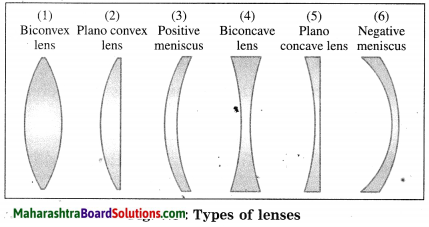[Note: Positive meniscus behaves as a convex lens as it is thicker in the middle than at the edges. Negative meniscus behaves as a concave lens as it is thicker at the edges than in the middle.]

Question 9.
In general, when a ray of light passes through a lens, there occurs a change in its direction of propagation. Why?
The working of a lens is similar to that of a triangular prism. When a ray of light passes through a lens, it is refracted twice: When entering the lens and when emerging from the lens. There is a change 5 in its direction of propagation every time and as both the changes occur in the same sense, the direction of propagation of the emergent ray is different from that of the incident ray.

Question 10.
State the rules used for drawing ray diagrams for the formation of an image by a convex lens.
Rules used for drawing ray diagrams for the formation of an image by a convex lens:
(1) When the incident ray is parallel to the principal axis, the refracted ray passes through the principal focus.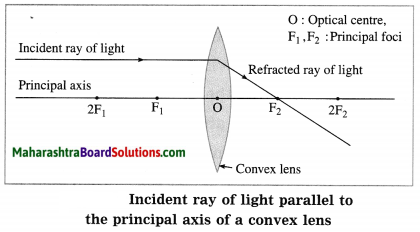(2) When the incident ray passes through the principal focus, the refracted ray is parallel to the principal axis.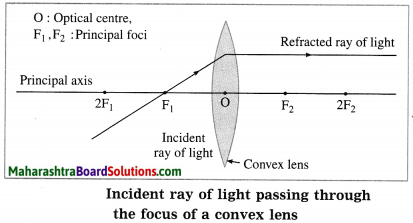(3) When the incident ray passes through the optical centre of the lens, it passes without changing its direction.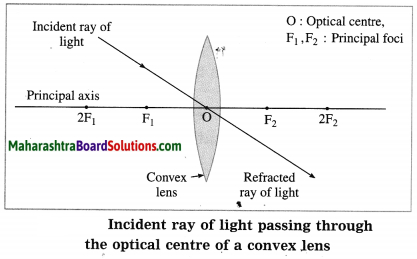Question 11.
In the case of a convex lens, show the path of the refracted ray when the incident ray of light (1) is parallel to the principal axis of the lens (2) passes through the focus of the lens (3) passes through the optical centre of the lens.
1.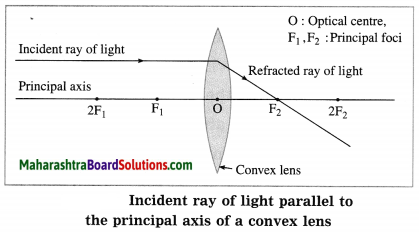2.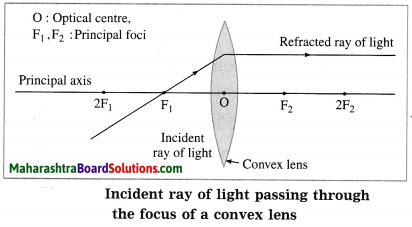3.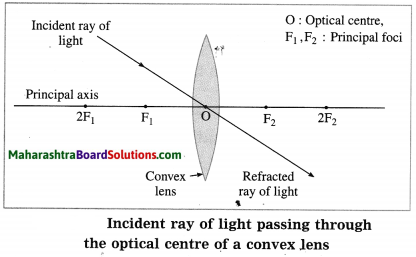Question 12.
Draw neat and well labelled ray diagrams for image formation by convex lens when an object is (1) at infinity (2) beyond 2F1 (3) at 2F1 (4) between F1 and 2F1 (5) at focus F1 (6) between focus F1 and optical centre O. Also, in each case, state the position, nature and size of the image relative to that of the object.
(1) Object at infinity: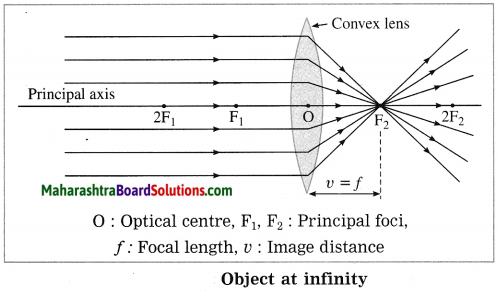In this case, the image is formed at focus F2 of the convex lens. It is real, inverted and highly diminished (point-sized).

(2) Object beyond 2F1: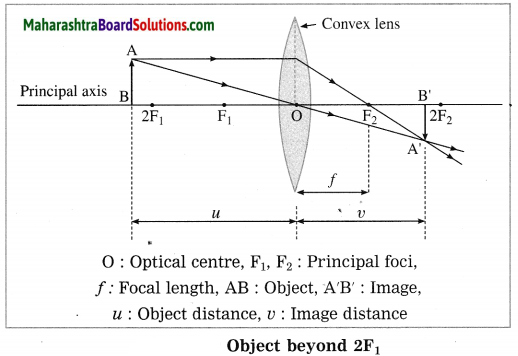In this case, the image is formed between F2 and 2F2. It is real, inverted and diminished.

(3) Object at 2F1: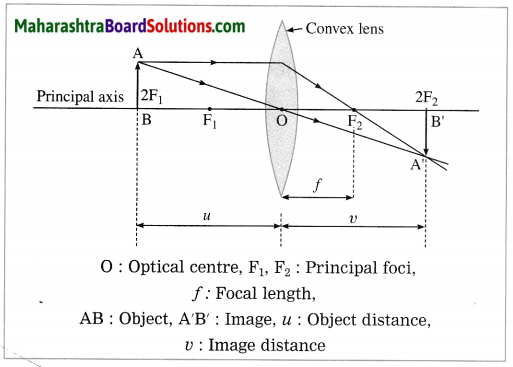In this case, the image is formed at 2F2. It is real, inverted and of the same size as that of the object.

(4) Object between F1 and 2F1: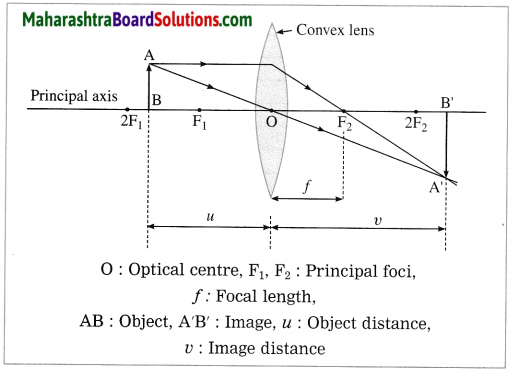In this case, the image is formed beyond 2F2. It is real, inverted and magnified (enlarged).

(5) Object at focus F1: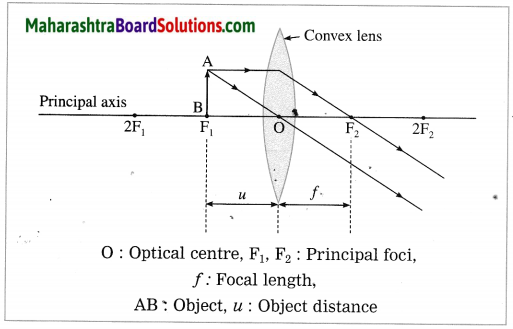In this case, the image is formed at infinity. It is real, inverted and infinitely large (highly magnified).

(6) Object between focus F1 and optical centre O: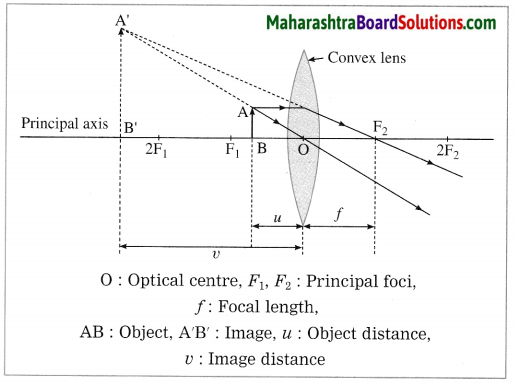In this case, the image is formed on the same side of the lens as the object. It is virtual, erect and larger than the object.
[Note: Here, the image is virtual. Hence, it is shown by a dotted line.]

Question 13.
Observe the following figure and complete the table: (March 2019)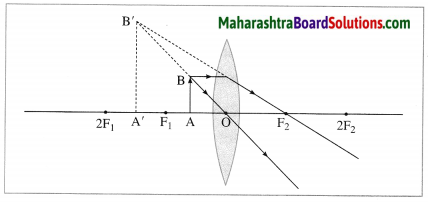Point Answer (i) Position of the object Between F1 and O (ii) Position of the image On the same side of the lens as the object (iii) Size of the image Very large (iv) Nature of the image Virtual and erect

Question 14.
At which position will you keep an object in front of a convex lens to get a real image smaller than the object? Draw a figure.
The object should be placed beyond 2F1.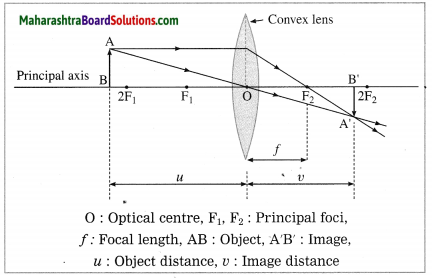Question 15.
State the rules used for drawing ray diagrams for the formation of an image by a concave lens.

1. When the incident ray is parallel to the principal axis, the refracted ray, when extended backwards, passes through the principal focus.
2. When the incident ray is directed towards the principal focus F2, the refracted ray is parallel to the principal axis.
3. When the incident ray passes through the optical centre of the lens, it passes without changing its direction.

Question 16.
State the characteristics of an image formed by a concave lens.
The image formed by a concave lens is always virtual, erect and smaller than the object. It is on the same side of the lens as the object. Generally, it is formed between the optical centre of the lens and the principal focus F1. If the object is at infinity, the image is a point image formed at F1.

Question 17.
In the case of image formation by a concave lens, what can you say about the position, nature and size of the image relative to the size of the object?
Image formation by a concave lens :
(1) If the object is at infinity, the image is formed at the focus of the lens, on the same side of the lens as the object. It is virtual, erect and much smaller than the object (point image).

(2) If the object is at any finite distance from the lens, the image is formed on the same side of the lens as the object and between the focus and the optical centre of the lens. It is virtual, erect and smaller than the object. The image distance is less than the object distance.

Question 18.
Draw a ray diagram to show image formation by a concave lens.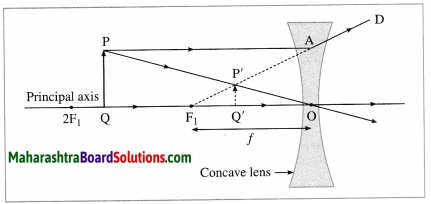PQ : Object
P’Q’ : Image (virtual, therefore shown by a dotted line),
O : Optical centre,
F1 : Principal focus,
f : Focal length of the lens
[Note: If in a Board examination, incomplete diagram (as shown below) is given, students should complete it and label its parts as shown in Figure.]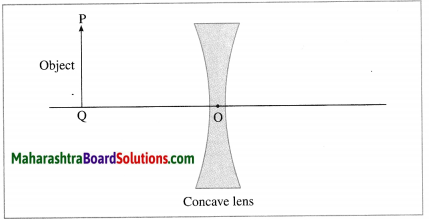Question 19.
State the Cartesian sign convention for refraction of light (image formation) by a lens.
Cartesian sign convention for refraction of light (image formation) by a lens:
In this case, the optical centre (O) of the lens is taken as the origin and the principal axis of the lens is taken as X-axis of the coordinate system.

(1) The object is always placed at the left of the lens.
All distances parallel to the principal axis are measured from the optical centre of the lens.
(2) All distances measured to the right of the origin are taken as positive while distances measured to the left of the origin are taken as negative.
(3) Distances measured perpendicular to and above the principal axis are taken as positive.
(4) Distances measured perpendicular to and below the principal axis are taken as negative.
(5) The focal length of a convex lens is positive and that of a concave lens is negative. (Fig. 7.21)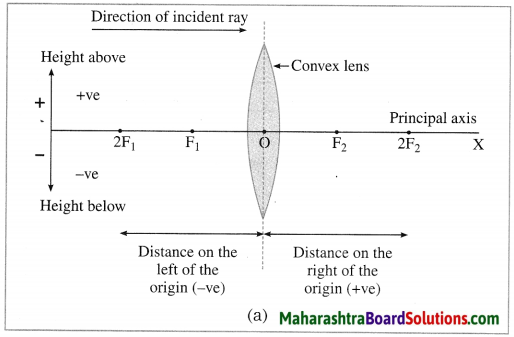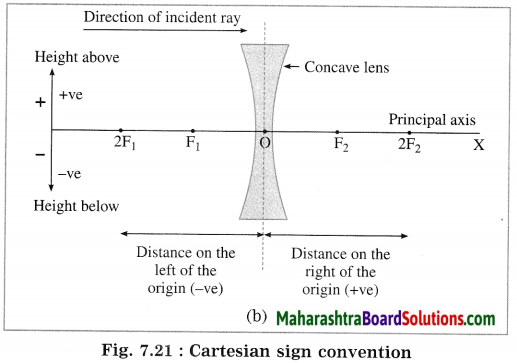Question 20.
(i) What is a lens formula? (ii) State it.
(i) The relationship between the object distance (u), image distance (v) and focal length (J) of a lens is called the lens formula.
(ii) It is 1𝑣1𝑢=1𝑓
[Note: The lens formula holds good for all values of u and v and is applicable to a convex lens as well as a concave lens. The sign convention for u, v and f must tie used in solving numerical examples.]

Question 21.
What is meant by the magnification produced by a lens? State the formulae for it.
The magnification (M) produced by a lens is the ratio of the height of the image (h2) to the height of the object (h1).
M = 21……….(1)
Also M = 𝑣( image distance )𝑢 (object distance) ……………(2)

Question 22.
When is the magnification produced by a lens (1) positive (2) negative?
The magnification produced by a lens is
(1) Positive when the image is virtual (as it is erect)
(2) Negative when the image is real (as it is inverted).

Question 23.
Express the magnification produced by a lens in terms of the focal length of the lens and (1) the object distance (2) the image distance.
Magnification (M) produced by a lens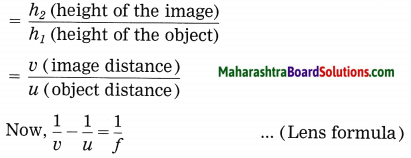where f is the focal length of the lens: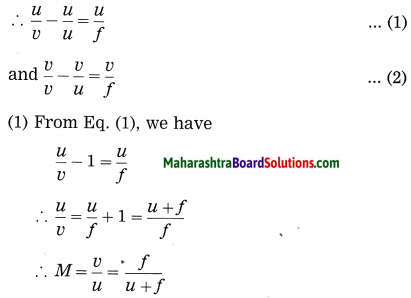(2) From eq. (2), we have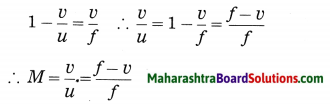Question 24.
An object is kept in front of a lens of focal length + 10 cm. Describe the nature of the image in the following cases: (1) The object” distance is 25 cm. (2) The object distance is 5 cm.
Since, the focal length of the lens ( +10 cm) is positive, it is a convex lens.
(1) If an object is kept at 25 cm from the lens, the image will be real, inverted and smaller than the object.
(2) If an object is kept at 5 cm from the lens, the image will be virtual, erect and larger than the object.

Question 25.
Anu and Anand have concave and convex lenses respectively. They took lenses in sunlight and tried to burn two pieces of paper of equal areas and temperature. State which lens will burn the paper. Give the reason. Explain with the help of a diagram, why the other paper did not burn.
(1) The convex lens will burn the paper. See Fig. 7.22 for reference. The ray of sunlight will converge at the principal focus of the lens. Hence, if the paper is held at the focus, it will burn due to concentration of heat energy.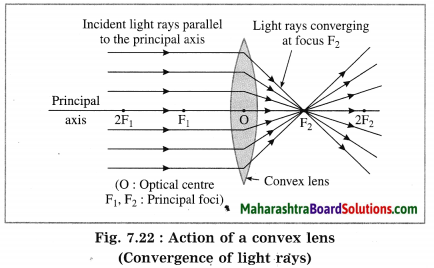(2) The paper held in front of the concave lens, will not burn. For reference, see Fig. 7.23. The concave lens will diverge the rays of sunlight falling on it. Hence, the paper will not burn.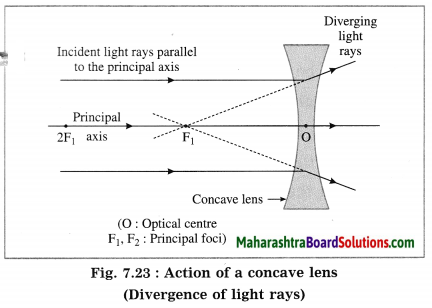Question 26.
To obtain a magnified real image of a small film strip, which type of lens is used? Where is the film strip placed to obtain the image on the screen?
To obtain a magnified real image of a small film strip, a convex lens is used. The film strip is placed between F1 and 2F1 and the screen is placed on the other side of the lens.

Question 27.
When an object of height 2 cm is placed in front of a convex lens, the height of the image is found to be 3 cm. State the nature and position of the image giving reason.
When an object is placed between the optical centre and the principal focus of a convex lens, the image formed by the lens is virtual and larger than the object. When an object is placed between F1 and 2Fthe image formed by the lens is real and larger than the object.

In the above case, if the image is virtual, it will be erect and on the same side of the lens as that of the object. If the image is real, it will be inverted and beyond 2F2 on the other side of the lens with respect to the object.

Question 28.
You are given a lens which gives a virtual, erect and enlarged image. What type of lens is it?
Since the lens gives a virtual, erect and enlarged image, it must be a convex lens.

Question 29.
When an object of height 3 cm is placed in front of a concave lens, the height of the image is found to be 6 cm. State, giving the reason, whether the given statement is true or false.
When an object is placed in front of a concave lens, the image formed by the lens is always smaller than the object. In the statement given in the question, the height of the image is reported as greater than that of the object. Hence, the statement given in the question is false.

Question 30.
State two uses of a concave lens.

1. A concave lens is used to correct myopia (nearsightedness).
2. In some optical instruments, a combination of a concave lens and a convex lens is used.

Question 31.
State two uses of a convex lens.
A convex lens is used (1) to read words in small print (2) to correct hypermetropia (Far-sightedness).

Question 32.
An object is kept in front of a lens of j focal length – 20 cm. Describe the nature of the image when the object distance is 25 cm.
Since the focal length of the lens (- 20 cm) is negative, it is a concave lens.
If an object is kept at 25 cm from the lens, the image will be virtual, erect and smaller than the object.
[Note: The nature of the image is independent of the object distance as it is a concave lens.]

Question 33.
An object is placed in front of a convex lens of focal length 20 cm. If the object distance is changed from 60 cm to 40 cm, what can you say about the size of the image relative to that of the object?
In this case, the focal length (f) of the lens is 20 cm.
∴ 2f = 40 cm.
When the object distance is 60 cm (which is greater than 2f), the image will be smaller than the object. When the object distance becomes 40 cm (which is equal to 2f), the image will be of the same size as that of the object.

Question 34.
What is the power of a lens?
The capacity of a lens to converge or diverge incident rays is called its power. The power (P) of a lens is the inverse of the focal length (f) of the lens.
P = 1𝑓

Question 35.
What is the unit of power of a lens? Define it.
The unit of power of a lens is the dioptre (D).
One dioptre is the power of a lens whose focal length is one metre.
1 dioptre (D) = 11 metre (m)
[Note: The dioptre, the SI unit of power of a lens, is denoted by D.]

Question 36.
What is the sign of the power of (i) a convex lens (ii) a concave lens?
The power of a convex lens is positive while that of a concave lens is negative.

Question 37.
If there is an increase or decrease in the focal length of a lens, what will be the effect on the power of the lens?
The power of a lens is the inverse of its focal length. Hence, if there is an increase in the focal length of a lens, the power of the lens will decrease accordingly. Similarly, if there is a decrease in the focal length of a lens, the power of the lens will increase accordingly.

Question 38.
If two lenses of focal lengths f1 and f2 are kept in contact with each other, state the formula for the focal length of the combination. If P1 and P2 are the powers of these lenses, state the formula for the power of the combination.
If two lenses of focal lengths f1 and f2 are kept in contact with each other, the focal length (f) of the combination is given by 1𝑓=1𝑓1+1𝑓2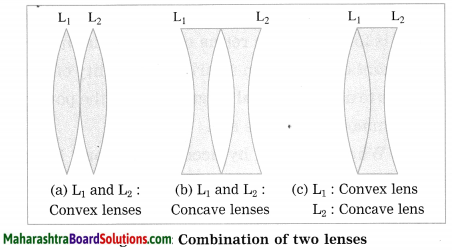[Two lenses kept in contact with each other]
If P1 and P2 are the powers of these lenses, the power (P) of the combination is given by P = P1 + P2.
[Note: The figures are given only for reference.]

Question 39.
Draw a neat labelled diagram to show the structure of the human eye.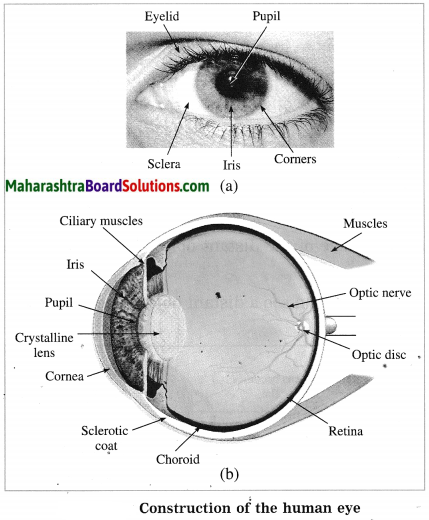Question 40.
What is cornea?
The cornea is a thin and transparent cover (membrane) on the human eye through which light enters the eye. Maximum refraction of light rays entering the eye occurs at the cornea.

Question 41.
What is iris?
The iris is a dark fleshy screen (muscular diaphragm) behind the cornea in the human eye. Its colours are different for different people.

Question 42.
What is pupil?
The pupil is a small circular opening of changing diameter at the centre of the iris in the human eye.

Question 43.
What is the use of the pupil in the human eye?
The pupil in the human eye is useful for controlling and regulating the amount of light entering the eye. The pupil contracts in the presence of too much light and dilates when light is insufficient, thus changing the amount of light entering the eye.

Question 44.
With reference to the functioning of the pupil in the human eye, what is adaptation?
The tendency of the pupil in the human eye to adjust the opening for light, depending on the intensity of incident light, to control and regulate the amount of light entering the eye is called adaptation.

Question 45.
What is the shape and the size of the human eyeball?
The human eyeball is approximately spherical in shape with a diameter of about 2.4 cm.

Question 46.
Name the part of the human eye that forms a transparent bulge on the surface of the eyeball.
The cornea forms a transparent bulge on the surface of the eyeball.

Question 47.
Which part of the human eye is located just behind the pupil?
A transparent biconvex crystalline lens is located just behind the pupil in the human eye.

Question 48.
What is retina?
The retina is a light sensitive screen consisting of a delicate membrane with a large number of light sensitive cells.

Question 49.
What is the nature of the eye lens and what does the eye lens do?
The eye lens is a double convex transparent crystalline lens, just behind the pupil. The eye lens provides small adjustment of focal length to form a real and inverted sharp image on the retina.

Question 50.
What happens when light falls on the retina?
When light falls on the retina, light sensitive cells of the retina are activated. They generate electrical signals which are passed by optic nerves to the brain. The brain interprets the signals and processes the information such that we perceive the object as it is.

Question 51.
What are ciliary muscles?
The muscles which hold the eye lens in its position, and bring about changes in the shape (curvature) of the eye lens, and hence of focal length are known as ciliary muscles.

Question 52.
What is the focal length of the eye lens of a normal eye in relaxed position of eye muscles?
The focal length of the eye lens of a normal eye in relaxed position of eye muscles is about 2 cm.

Question 53.
Where does the second focal point of the eye lens of a normal eye in relaxed position of eye muscles lie?
The second focal point of the eye lens of a normal eye in relaxed position of eye muscles lies on the retina.

Question 54.
What is meant by power of accommodation of the eye?
The ability of the eye lens to adjust its focal length is called the power of accommodation of the eye.

Question 55.
Explain the term power of accommoda¬tion of the eye.
(OR)
Write a short note on the power of accommodation of the eye.
Power of accommodation of the eye: The eye lens is held in its position by the ciliary muscles. When we look at a nearby object, the ciliary muscles compress the eye lens so that it becomes rounded. Hence, the focal length of the eye lens decreases. Therefore, the image is formed on the retina of the eye and hence the nearby object is seen clearly.

When we look at a distant object, the ciliary muscles relax so that the eye lens becomes flat. Hence, the focal length of the eye lens increases. Therefore, the image is formed on the retina of the eye and hence the distant object is seen clearly. This ability of the eye lens to adjust its focal length is called the power of accommodation of the eye.

Question 56.
What is meant by accommodation? How is it brought about?
The process of focusing the eye on objects at different distances is called accommodation. It is brought about by changing the curvature of the f elastic eye lens making it thinner or thicker.

Question 57.
The human eye is very similar to a photographic camera. The figure given shows the main parts of a photographic camera. Now answer the following questions: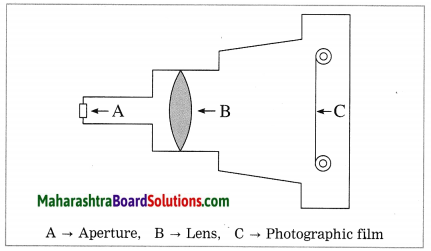(1) Name the parts of the human eye similar to the following parts of the photographic camera :
(a) Photographic film (b) Aperture.
(2) State one difference between the human eye lens and camera lens.
(3) Name the muscles which adjust the curvature of the eye lens.
(4) Which phenomenon of light is responsible for the working of the eye?
(1) (a) The retina in the human eye is similar to the photographic film in a camera.
(b) The pupil in the human eye is similar to the aperture in a camera.
(2) In a photographic camera, the focal length of the lens changes when the position of the lens is changed. In the human eye, the focal length of the eye lens is changed by the ciliary muscles and the distance of the image from the eye lens is fixed.
(3) The ciliary muscles adjust the curvature of the eye lens.
(4) The refraction of light is responsible for the working of the eye.

Question 58.
Have you seen a photographic camera in which a film is used? Compare the human eye with it. State similarities between them. State the points of difference between them.
Yes, we have seen a photographic camera in which a film is used.
Cameras, in general, have various shapes and sizes. Some cameras are much bigger than the human eye while some are smaller than the human eye. Here we shall consider a simple camera.

Similarities: In the case of a camera as well as the human eye, it is possible to control the amount of incoming light with the help of a diaphragm and an aperture. Both use a convex lens for focusing. The photographic film in a camera is coated with a photosensitive material. The retina in the eye consists of a large number of light sensitive cells. The photographic film in a camera is processed using chemicals and then prints (photographs) can be obtained using the appropriate paper.

In the human eye, the electrical signals generated by light sensitive cells are passed by optic nerves to the brain which interprets them.

Differences: Cameras come in a variety of sizes and shapes unlike the human eye. Unlike the human eye, a wide variation in exposure time is possible in the case of cameras. The human eye is sensitive in the visible region (red to violet) of the electromagnetic spectrum, while a much wider range of the electromagnetic spectrum can be covered with cameras designed for specific J purposes. In comparison with the human eye, a wider view and range can be covered by a camera.

In comparison with the human eye, a wider intensity (of light) range can be covered with a camera. The retina is indispensable in the human eye, while cameras without a photographic film have been designed with the help of photosensitive materials and are in current use.

[Note: With advances in technology, improved cameras are designed all the time, and the list of differences between the human eye and a camera in general would be practically endless.]

Question 59.
What is meant by the minimum distance of distinct vision?
The minimum distance from the normal eye, at which an object is clearly visible without stress on the eye is called the minimum distance of distinct vision.

Question 60.
Explain the term minimum distance of distinct vision.
(OR) Write a short note on distance of distinct vision.
Minimum distance of distinct vision; Though the focal length of the eye lens is adjustable, it cannot be decreased below a certain limit. Hence, if an object is very close to the eye, it cannot be seen clearly. For a normal human eye, the minimum distance from the eye at which an object is clearly visible without stress on the eye, is called the minimum distance of distinct vision. For the normal human eye, it is 25 cm.

Question 61.
State four reasons related to problems of vision.
Problems of vision are related to (i) weakening of ciliary muscles (ii) change in the size of the eyeball (iii) irregularities on the surface of cornea (iv) formation of a membrane over the eye lens.

Question 62.
What is myopia or nearsightedness? What are the possible reasons of myopia? How is myopia corrected? Explain with diagrams.
Myopia or nearsightedness is the defect of vision in which a human eye can see nearby objects distinctly but is unable to see distant objects clearly as they appear indistinct.
In this case the image of a distant object is formed in front of the retina instead of on the retina. [Figs. 7.29 (a), 7.29 (b)]

Possible reasons of myopia: (1) The curvature of the cornea and the eye lens increases. The muscles near the lens cannot relax so that the converging power of the lens remains large. (2) The distance between the eye lens and the retina increases as the eyeball elongates.

Myopia is corrected using a suitable concave lens. Light rays are diverged by the concave lens before they strike the eye lens. A concave lens of proper focal length is chosen to produce the required divergence. Hence, after the converging action of the eye lens, the image is formed on the retina. [Fig. 7.29 (c)]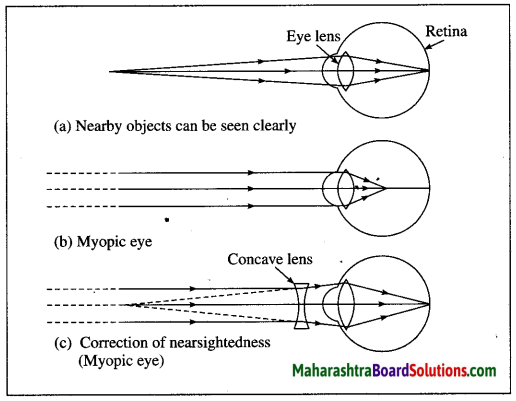Question 63.
Observe the following diagram and answer the questions.
(a) Which eye defect is shown in this diagram?
(b) What are the possible reasons for this eye defect?
(c) How is this defect corrected? Write it in brief.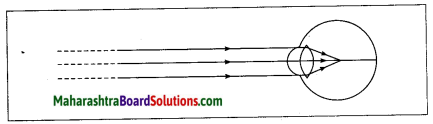(a) Myopia or Nearsightedness

(b) Possible reasons for the defect:
(i) The curvature of the cornea and the eye lens increases. The muscles near the lens cannot relax so that the converging power of the lens remains large.
(ii) The eyeball elongates so that the distance between the lens and the retina increases.

(c) Correction of the defect: This defect can be corrected using spectacles with concave lenses.
A concave lens diverges the incident rays and these diverged rays can be converged by the lens in the eye to form an image on the retina.

Question 64.
What is the sign of the power of the lens used to correct myopia?
The power of the lens used to correct myopia is negative.
[Note: It is a concave lens. Negative focal length Negative power.]

Question 65.
In a Std. X class, out of 40 students, 10 students use spectacles, 2 students have ( positive power and 8 students have negative power of lenses in their spectacles.
(1) What does the negative power indicate?
(2) What does the positive power indicate?
(3) Generally which type of spectacles do most of the students use?
(4) What defect of eyesight do most of the students suffer from?
(5) Give two possible reasons for the above defect.
(1) The negative power indicates a concave lens or myopia.
(2) The positive power indicates a convex lens or hypermetropia.
(3) Generally, most of the students use spectacles with concave lenses.
(4) Most of the students suffer from myopia.
(5) Two possible reasons for myopia:

1. The curvature of the cornea and the eye lens increases. The muscles near the lens cannot relax so that the converging power of the lens remains large.
2. The distance between the eye lens and the retina increases as the eyeball elongates.

Question 66.
What is hypermetropia or farsightedness? What are the possible reasons of hypermetropia? How is hypermetropia corrected? Explain with figures.
Hypermetropia or farsightedness is the defect of vision in which a human eye can see distant objects clearly but is unable to see nearby objects clearly.
In this case the image of a nearby object would fall behind the retina instead of on the retina.
[Figs. 7.31 (a), 7.31 (b)]

Possible reasons of hypermetropia:
(1) Curvature of the cornea and the eye lens decreases. Hence, the converging power of the eye lens becomes less. (2) The distance between the eye lens and retina decreases (relative to the normal eye) and the focal length of the eye lens becomes very large due to the flattening of the eyeball.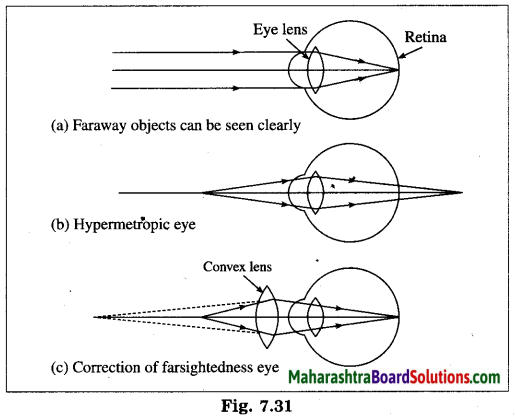Hypermetropia is corrected using a suitable convex lens. Light rays are converged by the convex lens before they strike the eye lens. A convex lens of proper focal length is chosen to produce the required convergence. Hence, after the converging action of the eye lens, the image is formed on the retina. [Fig. 7.31 (c)]

Question 67.
What is the sign of the power of the lens used to correct hypermetropia?
The power of the lens used to correct hypermetropia is positive.
[Note: It is a convex lens. Positive focal length ∴ Positive power.]

Question 68.
Given below is a diagram showing a defect of human eye.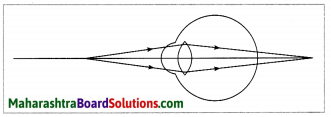Study it and answer the following questions:
(1) Name the defect shown in the figure. (Practice Activity Sheet – 3)
(2) Give two possible reasons for this defect of eye in human beings.
(3) Name the type of lens used to correct the eye defect.
(4) Draw a labelled diagram to show how the defect is rectified by using the lens.
A lens having both spherical surfaces puffed up outwards is called a convex lens or double convex lens or biconvex lens. It is thicker in the middle than at the edges.
[Note: A convex lens is also called a converging lens.]

Question 69.
Observe the following figures and complete the table. (Practice Activity Sheet – 1)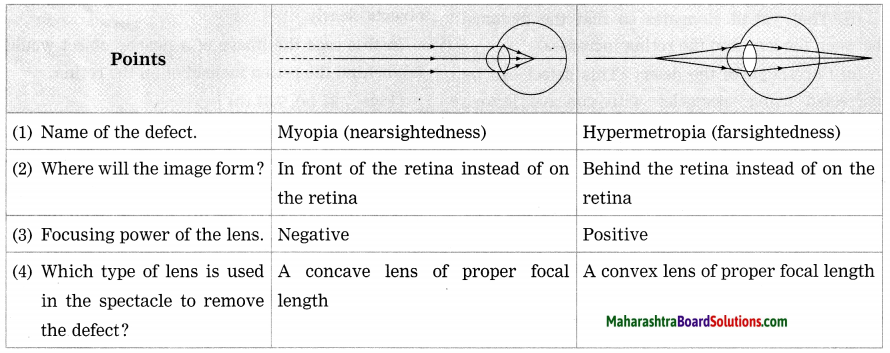Question 70.
What is presbyopia? State the reason for this defect. How is presbyopia corrected?
Presbyopia is the defect of vision in which aged people find it difficult to see nearby objects comfortably and clearly without spectacles.

Reason of presbyopia: The power of accommodation of eye usually decreases with ageing. The muscles near the eye lens lose their ability to change the focal length of the lens.
Therefore, the near point of the eye lens shifts farther from the eye.

This defect is corrected using a convex lens of appropriate power. The lens converges light rays before they fall on the eye lens such that the action of the eye lens forms the image on the retina.

Question 71.
What is a bifocal lens?
A bifocal lens is a lens of which the upper part is a concave lens to correct myopia and the lower part is a convex lens to correct hypermetropia.

[Note: A person suffering from myopia as well as hypermetropia, uses a bifocal lens. Nowadays, the defects of vision such as myopia and hypermetropia can be corrected using contact lenses or by laser surgery.]

Question 72.
(A) Anil cannot see the blackboard writing clearly, but he can see nearby objects clearly.
(i) What is the eye defect he is suffering from?
(ii) How is it corrected?
(B) Anil’s uncle cannot see nearby objects clearly, but he can see distant objects clearly.
(i) What is the eye defect he is suffering from?
(ii) How is it corrected?
(A) Anil cannot see the blackboard writing clearly, but he can see nearby objects clearly.
(i) This defect is called myopia (nearsightedness).
(ii) It is corrected using spectacles having concave lenses of appropriate power.

(B) Anil’s uncle cannot see nearby objects clearly, but he can see distant objects clearly.
(i) This defect is called hypermetropia (farsightedness).
(ii) It is corrected using, spectacles having convex lenses of appropriate power.

Question 73.
When are bifocal lenses used in spectacles?
When a person cannot see nearby objects as well as distant objects clearly, bifocal lenses are used in spectacles.

Question 74.
Aniket from Std. X uses spectacles. The power of the lenses in his spectacles is -0.5 D. Answer the following questions:
(1) State the type of’ lenses used in his spectacles.
(2) Name the defect of vision Aniket is suffering from.
(3) Find the focal length of the lenses used in his spectacles.
(1) Concave lenses are used in the spectacles used by Aniket.
(2) Aniket is suffering from myopia (near-sightedness).
(3) Focal length of the lenses used in his spectacles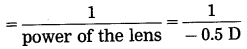= -2 m (Concave lens ∴ Minus sign)

Question 75.
Sunita from Std. X uses spectacles. Her spectacle number is -1.5 D. Answer the following questions:
(1) Name the defect of eye from which she is suffering.
(2) What type of lens is she using?
(3) Find the focal length of the lens.
(1) Myopia.
(2) Concave.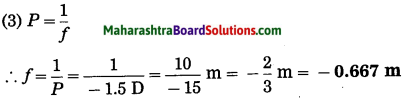(Concave lens ∴ minus sign)
This is the focal length of the lens.

Question 76.
Surabhi from Std. X uses spectacles. The power of the lenses in her spectacles is 0.5 D. Answer the following questions from the given information: (March 2019)
(i) Identify the type of lenses used in her spectacles.
(ii) Identify the defect of vision Surabhi is suffering from.
(iii) Find the focal length of the lenses used in her spectacles.
(i) Convex lenses are used in the spectacles used by Surabhi.
(ii) Surabhi is suffering from hypermetropia (farsightedness).
(iii) Focal length of the lenses used in her spectacles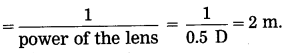Question 77.
My grandfather uses a bifocal lens in his spectacles. Explain why.
In old age, people usually suffer from both myopia and hypermetropia. Therefore, they need spectacles having bifocal lenses.

The upper part of a bifocal lens is a concave lens to correct myopia. The lower part of a bifocal lens is a convex lens to correct hypermetropia.

Question 78.
State uses of concave lens.

1. Concave lenses are used for proper working of medical equipment, scanner, CD player – the instruments that employ laser rays.
2. One or more concave lenses are used in a small safety device, fitted in the peep hole in a door, due to which we can see a large area outside the door.
3. Concave lenses are used in spectacles to correct nearsightedness (myopia).
4. A concave lens is used to spread light emitted by the small bulb in a torch over a wide area.
5. A concave lens is used in front of the eyepiece or inside the eyepiece fitted in a camera, telescope and microscope – the instruments employing convex lenses.

Question 79.
State uses of a convex lens.
Convex lenses are used in a simple microscope, compound microscope, refracting telescope, camera, projector, spectroscope, spectacles for correcting farsightedness (hypermetropia) and binoculars.

Question 80.
What is meant by the apparent size of an object? With a neat and labelled diagram, explain the relation between the apparent size of an object and the angle subtended by the object at the eye.
An object appears small or big depending upon the size of its image formed on the retina of the eye. The size of an object as perceived by the eye is called the apparent size of the object. Consider two objects of the same size, one held near the eye and the other away from the eye as shown in the following figure (Fig. 7.34). The nearby object (PQ) appears larger than the distant object (P1Q1).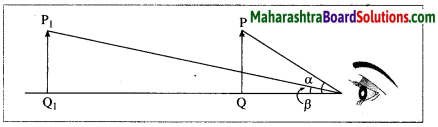Also, the angle a subtended by the nearby object at the eye is larger than the angle β subtended by the distant object at the eye. This shows that the apparent size of an object depends upon the angle subtended by the object at the eye. The greater the angle subtended by the object at the eye, the greater is the apparent size of the object. Similarly, the smaller the angle subtended by the object at the eye, the smaller is the apparent size of the object.

Question 81.
With a neat labelled diagram, explain the working of a simple microscope. State uses of a simple microscope.
(OR)
What does a simple microscope consist of? What is the order of magnification obtained by a simple microscope? What is a simple microscope used for?
A simple microscope consists of a convex lens of short focal length, usually fixed in a suitable frame with a handle or mounted on a stand.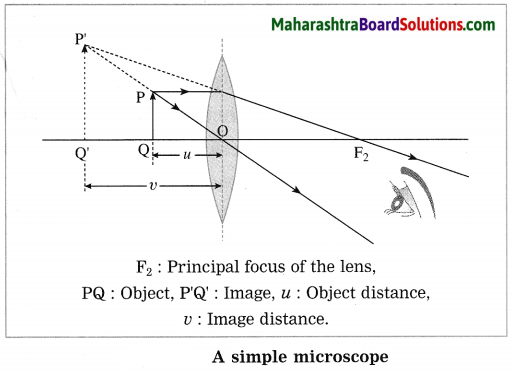The object is placed in front of the convex lens of short focal length such that the object distance is less than the focal length. The image is virtual and larger than the object. It is formed on the same side of the lens as the object.

A maximum magnification of about 20 can be obtained by a simple microscope. A simple microscope is used by watch repairers to observe small parts of a watch and by jewellers to examine ornaments. A simple microscope (also called a magnifying glass) is also used to read words in small print.

Question 82.
With a neat labelled diagram, explain the construction and working of a compound microscope.
Construction of a compound microscope: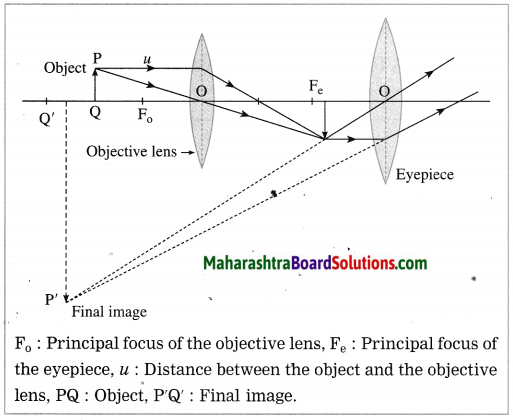(1) A compound microscope consists of a metal tube fitted with two convex lenses at the two ends. These lenses are called the objective lens (the lens directed towards the object) and the eyepiece (the lens directed towards the eye). Both the lenses are small in size, but the cross section of the objective lens is less than that of the eyepiece. The objective lens has a short focal length. The focal length of the eyepiece is more than that of the objective lens.

(2) The metal tube is mounted on a stand. The principal axes of the objective lens and the eyepiece are along the same line. The distance between the object and the objective lens can be changed with a screw. It is possible to change the distance between the objective lens and the eyepiece.

Working :
(1) The object to be observed is illuminated and placed in front of the objective lens, slightly beyond the focal length of the objective lens. Its real, inverted and enlarged image is formed by the objective lens on the other side.

(2) This intermediate image lies within the focal length of the eyepiece. It serves as an object for the eyepiece. The eyepiece works as a simple microscope. The final image is virtual, highly enlarged and inverted with respect to the original object. It can be formed at the minimum distance of distinct vision from the eyepiece. The final image is observed by keeping the eye close to the eyepiece.

Question 83.
State two uses of a compound microscope.
Uses of a compound microscope:

1. It is used to observe blood corpuscles, plant and animals cells, microorganisms like bacteria, etc.
2. It is used in a pathological laboratory to observe blood, urine, etc.
3. It is a part of a travelling microscope used for measurement of very small distance.

Question 84.
What will happen if in a compound microscope, the objective lens is large in size and has a focal length?
If the objective lens of a compound microscope is large in size, in addition to the light coming from an object, other unwanted light will be incident on the objective lens. Hence, the image will not be seen clearly. If the objective lens has a large focal length, the magnification produced by it will be less.

Question 85.
(a) In which type of microscope do you find the lens arrangement as shown in the following diagram? (Practice Activity Sheet – 1)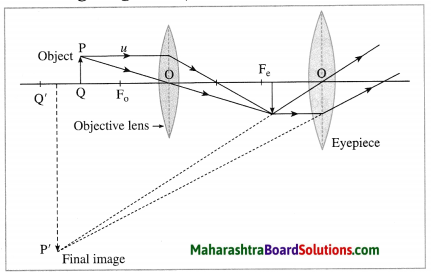(b) Write in brief, the working of this microscope.
(c) Where is this microscope used?
(a) Compound microscope.

(b)
An object appears small or big depending upon the size of its image formed on the retina of the eye. The size of an object as perceived by the eye is called the apparent size of the object. Consider two objects of the same size, one held near the eye and the other away from the eye as shown in the following figure (Fig. 7.34). The nearby object (PQ) appears larger than the distant object (P1Q1).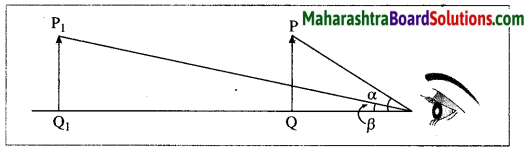Also, the angle a subtended by the nearby object at the eye is larger than the angle β subtended by the distant object at the eye. This shows that the apparent size of an object depends upon the angle subtended by the object at the eye. The greater the angle subtended by the object at the eye, the greater is the apparent size of the object. Similarly, the smaller the angle subtended by the object at the eye, the smaller is the apparent size of the object.

(c) A simple microscope is used by watch repairers to observe small parts of a watch and by jewellers to
examine ornaments. A simple microscope (also called a magnifying glass) is also used to read words in small print.

Question 86.
(i) Which type of microscope has the arrangement of lenses shown in the following figure?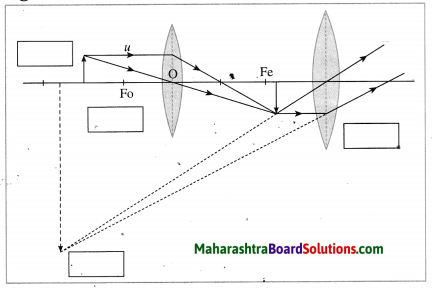(ii) Label the figure correctly.
(iii) Write the working of this microscope.
(iv) Where is this microscope used?
(v) Suggest a way to increase the efficiency of this microscope. (Practice Activity Sheet – 2)
(i) Compound microscope.

(ii)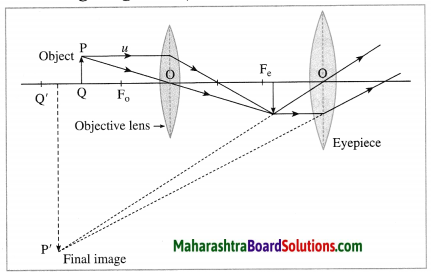(iii) Working:
(1) The object to be observed is illuminated and placed in front of the objective lens, slightly beyond the focal length of the objective lens. Its real, inverted and enlarged image is formed by the objective lens on the other side.

(2) This intermediate image lies within the focal length of the eyepiece. It serves as an object for the eyepiece. The eyepiece works as a simple microscope. The final image is virtual, highly enlarged and inverted with respect to the original object. It can be formed at the minimum distance of distinct vision from the eyepiece. The final image is observed by keeping the eye close to the eyepiece.

(iv)

1. It is used to observe blood corpuscles, plant and animals cells, microorganisms like bacteria. etc.
2. It is used in a pathological laboratory to observe blood, urine, etc.
3. It is a part of a travelling microscope used for measurement of very small distance.

(v) Lenses with appropriate focal lengths should be selected.

Question 87.
State the use of a telescope.
A telescope is used to observe a distant object such as mountain, moon, planet, star in the magnified form.

Question 88.
Observe the following figure and answer the questions. (Practice Activity Sheet – 3)
(a) Which optical instrument shows arrangement of lenses as shown in the figure?
(b) Write in brief the working of this optical instrument.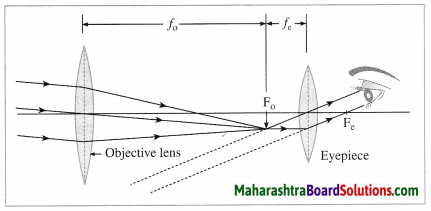(c) How can we get different magnifications in this optical instrument?
(d) Draw the figure again and labelled it properly.
(a) Refracting telescope.

(b) working: When the objective lens is pointed towards the distant object to be observed, the rays
of light from the distant object, which are almost parallel to each other. pass through the objective lens. The objective lens collects maximum amount of light as it is large in size. It forms a real, inverted and diminished image in the focal plane of the objective lens. Now, the position of the eyepiece is adjusted such that this image falls just within the focal length of the eyepiece and serves as the object for the eyepiece which works as a simple microscope.

The final image is highly magnified, virtual, on the same side as that of the object and inverted with respect to the original object. The final image can be observed by keeping the eye close to the eyepiece. If the image formed by the objective lens lies in the focal plane of the eyepiece, the final image is formed at infinity.

(c) We can get different magnifications by using the eyepiece with different focal lengths.

(d)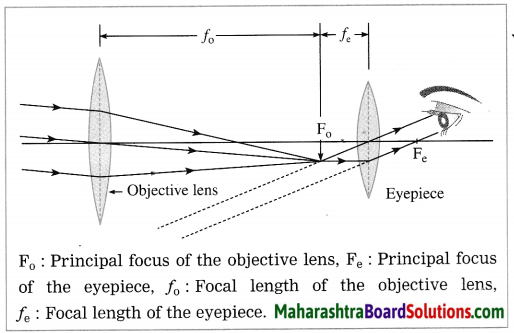Question 89.
What is persistence of vision? Give one example of persistence of vision.
Persistence of vision: We see an object when its image is formed on the retina. The image disappears when the object is removed from our sight. But this is not instantaneous and the image
remains imprinted on the retina for about 116 th of a second after the removal of the object.

The sensation on the retina persists for a while. This effect is known as the persistence of vision. It is due to persistence of vision that we continue to see the object in its position for about 116 th of a second after it is removed.
Example: When a burning stick of incense is moved fast in a circle, a circle of red light is seen.

Question 90.
Name two devices whose working is based on the phenomenon of persistence of vision.
(OR)
Name any two applications based on persistence of vision.
The working of a television set and motion picture is based on the phenomenon of persistence of vision.
[Note: These are the examples of persistence of vision in daily life.

Question 91.
How is the phenomenon of persistence of vision used in motion pictures?
In motion pictures, photographs of a moving object are taken at the rate of more than sixteen pictures per second. These photographs are projected on the screen at the same rate.
Each picture is slightly different from the other. As a result of persistence of vision, we get the impression of observing the object in continuous motion.

Question 92.
Name the two types of light sensitive cells present in the retina of the human eye. What are their functions?
(1) The retina of the human eye contains a large number of light sensitive cells. These cells are of two shapes : (i) rods and (ii) cones.
(2) The rod-like cells respond to the intensity of light.
(3) The conical cells respond to various colours of light. They respond differently to red, green and blue colours. They do not respond to faint light.

Question 93.
When do you say that a person is colour blind?
When a person is unable to distinguish between certain colours, he is said to be colour blind.
[Note: (i) Except for being colour-blind, their eyesight is normal, (ii) Rod-shaped cell ≡ rod-like cell, cone-shaped cell = Conical cell.]

Question 94.
Explain the perception of colour in the human eye.
(OR)
Explain in short perception of colour.
(OR)
Write a note on perception of colour.
(1) In nature we firld objects of various colours. Perception of colour means to be able to respond to colour.
(2) We can distinguish between various colours due to perception of colour.
(3) The cone-shaped cells on the retina of the eye respond to the various colours when light is bright and communicate to the brain about the colours of the image formed on the retina. This gives us the proper idea about the colours of the object.
(4) If, in the retina of a person, the cone-shaped cells responding to certain specific colours are absent, the person is unable to distinguish between the colours. As a result, he lacks perception of colour.

Question 95.
What is colour-blindness?
(1) The retina of the human eye contains a large number of light sensitive cells. These cells are of two shapes : (i) rods and (ii) cones.
(2) The cone-shaped cells respond to various colours of light when light is bright.
(3) Thus, the perception of colour is due to the presence of the cone-shaped cells in the retina.
(4) In the retina of some persons, cone-shaped cells responding to certain specific colours are absent. Hence, these persons are unable to distinguish between certain colours, i.e., they are colour-blind. This defect is known as colour¬blindness.

Question 96.
Why are some persons colour-blind? What is the cause of this defect?
In the retina of some persons, cone-shaped cells responding to certain specific colours are absent. Hence, these persons are unable to distinguish between certain colours, i.e., they are colour-blind.

Question 97.
What are the difficulties faced by a colour-blind person?
(1) A colour-blind person cannot distinguish between different colours. For example, he cannot distinguish between red and green colours. Also he cannot distinguish between blue and green colours. Red and green, both appear grey. Since a colour¬blind person cannot distinguish between red and green colours, it is difficult for him to cross a road. There is a possibility of an accident while crossing a road.

(2) A colour-blind person cannot distinguish between two objects of different colours, which are otherwise identical, e.g., clothes.

(3) A colour-blind person may have an inferiority complex and hence may find it difficult to mix with other persons.

Give scientific reasons:

Question 1.
A convex lens is known as a converging lens.
When rays of light parallel to the principal axis of a convex lens pass through the lens, they converge to a point on the principal axis. Hence, a convex lens is known as a converging lens.

Question 2.
A concave lens is called a diverging lens.
When rays of light parallel to the principal axis of a concave lens pass through the lens, they appear to diverge from a point on the principal axis. Hence, a concave lens is called a diverging lens.

Question 3.
In old age, a bifocal lens is necessary for some persons.
(1) Some people, in old age, suffer from myopia (nearsightedness) as well as hypermetropia (farsightedness).
(2) Myopia is corrected using a concave lens of appropriate power. Hypermetropia is corrected using a convex lens of appropriate power. Therefore, they need a bifocal lens.

Question 4.
A person suffering from myopia (nearsightedness) uses spectacles of concave lenses.
(1) A person suffering from myopia can see nearby objects clearly as the image of a nearby object is formed on the retina, but cannot see distant objects clearly as the image of a distant object is formed in front of the retina instead of on the retina.

(2) A concave lens diverges the rays of light passing through it. When spectacles of concave lenses of appropriate power are used, the parallel rays coming from a distant object are diverged to proper extent before they are incident on the eye lens. Therefore, after the converging action of the eye lens, the image of a distant object is formed on the retina of the eye and hence the distant object can be seen clearly.

Question 5.
A person suffering from hypermetropia (farsightedness) uses spectacles of convex lenses.
(1) A person suffering from hypermetropia can see distant objects clearly as the image of a distant object is formed on the retina, but cannot see nearby objects clearly as the image of a nearby object would be formed behind the retina instead of on the retina.

(2) A convex lens converges the rays of light passing through it. When spectacles of convex lenses of appropriate power are used, the rays of light coming from a nearby object are converged to proper extent before they are incident on the eye lens. Therefore after the converging action of the j eye lens, the image of a nearby object is formed on j the retina of the eye and hence the nearby object | can be seen clearly.

Question 6.
You cannot enjoy watching a movie from a very short distance from the screen in a cinema hall.
(1) The less the distance between the screen in a cinema hall and the person watching the movie, the more is the intensity of light falling on the eye.
(2) This results in great contraction of the pupil of the eye causing a strain. Hence, you cannot enjoy watching a movie from a very short distance from the screen in a cinema hall.

Question 7.
The rays of light travelling through the optical centre of a lens pass without changing their path.
The portion of a lens near the optical centre is like a very thin slab of glass. Hence, the rays of light travelling through the optical centre of a lens pass without changing their path.

Question 8.
A convex lens converges the rays of light falling on it.

• A convex lens can be regarded as made of a very large number of portions of triangular prisms. The bases of these prisms are towards the central thicker portion of the lens.
• A ray of light passing through a prism bends towards its base. Hence, a convex lens converges the rays falling on it.

Question 9.
A concave lens diverges the rays of light falling on it.

• A concave lens can be regarded as made of a very large number of portions of triangular prisms. The bases of these prisms are towards the edges of the less, i.e, away from the central thinner portion of the lens.
• A ray of light passing through a prism bends towards its base. Hence, a concave lens diverges the rays of light falling on it.

Question 10.
When a burning stick of incense is moved fast in a circle, a circle of red light is seen.
The impression of the image on the retina lasts for about 116 th of a second after the removal of the object. If a burning stick of incense is moved at a rate of more than sixteen revolutions per second, we see a circle of red light due to persistence of vision.

Question 11.
Colour-blind persons are unable to distinguish between different colours.
(1) The cone-shaped cells in the retina of a person respond to colours. This makes the perception of colours possible.
(2) In the retina of colour-blind persons, cone-shaped cells responding to certain specific colours are absent. Hence, they are unable to distinguish between different colours.

Question 12.
It is risky to issue a driving license to a person suffering from colour-blindness.
A colour-blind person cannot distinguish between different colours. If a driver is colour-blind, he will not be able to distinguish between the colours of the signal and the colours on different sign boards. This will lead to an accident. Hence, it is risky to issue a driving license to a person suffering from colour-blindness.

Distinguish the following:

Question 1.
Real image and Virtual image.
Real image:

1. A real image is formed when the light rays starting from an object meet after reflection or refraction.
2. It can be projected on a screen.
3. It is inverted with respect to the object.

Virtual image:

1. A virtual image is formed when the light rays starting from an object (when extended backward) appear to meet after reflection or refraction.
2. It cannot be projected on a screen.
3. It is erect with respect to the object.

Question 2.
Simple microscope and Compound microscope.
Simple microscope:

1. In a simple microscope, only one convex lens is used.
2. In this case, the object is placed within the focal length of the convex lens.
3. Its magnifying power is much less than that of a compound microscope.
4. It is used to observe minute parts of a watch, to read words in small print, etc.

Compound microscope:

1. In a compound microscope, two convex lenses, objective and eyepiece, are used.
2. In this case, the object is placed beyond the focal length of the objective lens.
3. Its magnifying power is much greater than that of a simple microscope.
4. It is used to observe blood corpuscles, plant and animal cells, etc.

Question 3.
Compound microscope and Astronomical refracting telescope.
Compound microscope:

1. In a compound microscope, the focal length and cross section of the objective lens are respectively smaller than the focal length and cross section of the eyepiece.
2. In this case, to observe the object, the distance between the object and the objective lens is adjusted.
3. It forms a magnified image of a small object.
4. It is used to observe blood corpuscles, plant and animal cells, etc.

Astronomical refracting telescope:

1. In an astronomical refracting telescope, the focal length and cross section of the objective lens are respectively greater than the focal length and cross section of the eyepiece.
2. In this case, to observe the object, the distance between the objective lens and eyepiece is adjusted.
3. It forms a near image of a distant object.
4. It is used to observe sateulites, planets, stars, etc.

Question 4.
Simple microscope and Astronomical refracting telescope.
Simple microscope:

1. In a simple microscope, only one convex lens is used.
2. In this case, the object is placed within the focal length of the convex lens.
3. In this case, the image is erect.
4. It is used to observe minute parts of a watch, to read words in small print, etc.

Astronomical refracting telescope:

1. In an astronomical refracting telescope, two convex lenses, objective lens and eyepiece are used.
2. In this case, the object is far away from the objective lens.
3. In this case, the image is inverted.
4. It is used to observe satellites, planets, stars, etc.

Construction of a compound microscope:
(1) A compound microscope consists of a metal tube fitted with two convex lenses at the two ends. These lenses are called the objective lens (the lens directed towards the object) and the eyepiece (the lens directed towards the eye). Both the lenses are small in size, but the cross section of the objective lens is less than that of the eyepiece. The objective lens has a short focal length. The focal length of the eyepiece is more than that of the objective lens.(2) The metal tube is mounted on a stand. The principal axes of the objective lens and the eyepiece are along the same line. The distance between the object and the objective lens can be changed with a screw. It is possible to change the distance between the objective lens and the eyepiece.
Working:
(1) The object to be observed is illuminated and placed in front of the objective lens, slightly beyond the focal length of the objective lens. Its real, inverted and enlarged image is formed by the, objective lens on the other side.
(2) This intermediate image lies within the focal length of the eyepiece. It serves as an object for the eyepiece. The eyepiece works as a simple microscope. The final image is virtual, highly enlarged and inverted with respect to the original object. It can be formed at the minimum distance of distinct vision from the eyepiece. The final image is observed by keeping the eye close to the eyepiece.
Use: This microscope is used to observe blood cells, microorganisms, etc.

Question 1.
In a compound microscope, which lens has greater focal length?
In a compound microscope, the eyepiece has greater focal length.

Question 2.
Where do you place the object to be observed with a compound microscope?
In a compound microscope, the object to be observed is placed in front of the objective lens, slightly beyond the focus of the objective lens.

Question 3.
State which distance is adjusted to observe the object with a compound microscope.
To observe the object with a compound microscope, the distance between the object and objective lens is adjusted.

Question 4.
State the nature of the final image in compound microscope relative to the object.
In a compound microscope, the final image is highly enlarged, inverted and virtual relative to the object.

Question 5.
State the use of a compound microscope.
A compound microscope is used to observe blood cells, microorganisms, etc.

Fill in the blanks for a convex lens:

Question 1.

 f (m) 0.2 —————– 0.1 P (D) ————— 2 ——————

[P (D) = 1𝑓(m)]

 f (m) 0.2 0.5 0.1 P (D) 5 2 10

Question 2.

 h1 (cm) —————- 5 10 h2 (cm) -30 -20 —————- M -2 —————– -0.5

[M = 21]

 h1 (cm) 15 5 10 h2 (cm) -30 -20 -5 M -2 -4 -0.5

Solve the following numerical problems:

Problem 1.
An object Is kept at 60 cm in front of a convex lens. Its real image is formed at 20 cm from the lens. Find the focal length or the lens.
Solution:
Data: Convex lens, u = -60 cm,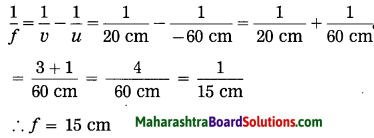The focal length of the lens = 15 cm.

Problem 2.
The focal length of a convex lens is 20 cm. If an object of height 2 cm is placed at 30 cm from the lens, find (i) the position and nature of the Image (ii) the height of the image (iii) the magnification produced by the lens.
Solution:
Data: Convex lens, f = 20 cm,
u = -30 cm, h1 = 2 cm, v = ?, h2 = ?, M = ?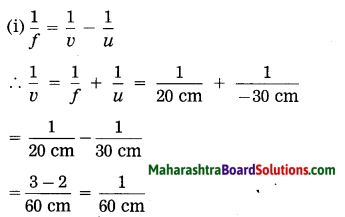The image will be formed at 60 cm from the lens and on the other side of the lens with respect to the object. It is a real image.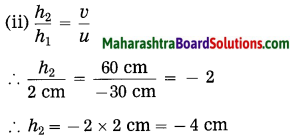h2 is negative. This shows that the image is inverted.
The height of the image -4 cm.
(iii) M = 21=4cm2cm = -2
M is negative, indicating that the image is inverted.
The magnification produced by the lens = -2.

Problem 3.
When a pm of height 3 cm is fixed at 10 cm from a convex lens, the height or the virtual image formed is 12 cm. Find the focal length of the lens.
Solution:
Data: Convex lens, h1 =3 cm,
h2 = 12 cm (virtual image), u = -10 cm, f = ?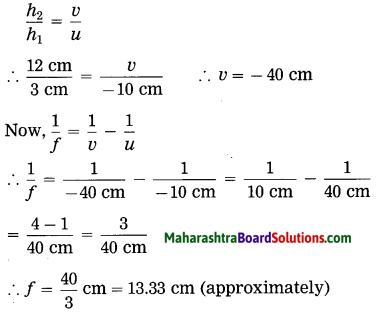The focal length of the lens = 13.33 cm [approximately]

Problem 4.
At what distance from a convex lens of focal length 2.5 m should a boy stand so that his image is half his height?
Solution:
Data: Convex lens, f= 2.5 m,
M= –12, u=?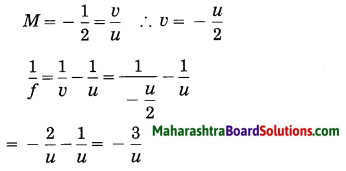∴ u = -3f = -3 × 2.5 m = -7.5 m
This is the object distance.
The boy should stand at 7.5 m from the convex lens so that his image is half his height.

Problem 5.
A convex lens forms a real image or a pencil at a distance of 40 cm from the lens. The image formed is of the same size as the object. Find the focal length and power of the lens. At what distance is the pencil placed from the lens?
Solution:
Data: Convex lens, v = 40 cm,
M = -1, f = ?, h1 = ?, u = ?
M = = -1 = 𝑣𝑢
∴ u = -v = -40 cm (object distance)
The pencil is placed at 40 cm from the convex lens.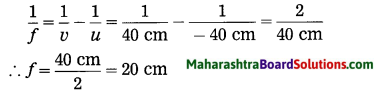The focal length of the lens 20 cm.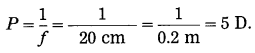The power of the lens = 5 D.

Problem 6.
A spherical lens is used to obtain an image on a screen. The size of the image is four times the size of the object. What is the type of lens and at what distance is the screen placed from the lens?
Solution:
Data: M = -4, type of lens? v = ?
As the image formed by the lens is obtained on a screen, it is a real image. The lens is, therefore, a convex 1ens.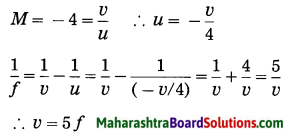The distance of the screen from the lens = 5f.

Problem 7.
An object of height 5 cm Is held 20 cm away from a converging lens of focal length 10 cm. Find the position, nature and size of the image formed.
Solution:
Data: Converging lens (convex lens),
f = 10 cm, h1 = 5 cm, u = -20 cm, v = ?, h2 = ?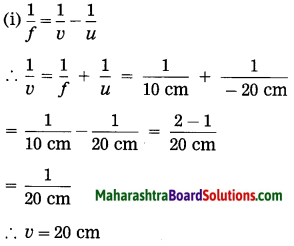The image is real and inverted. it is formed at 20 cm from the lens and on the other side of the lens relative to the object.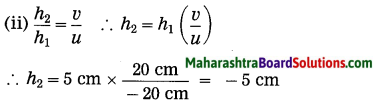The height of the image, h2 = -5 cm
Thus, it is numerically the same as the height of the object.

Problem 8.
An object is placed at 10 cm from a convex lens of focal length 12 m. Find the position and nature of the image.
Solution:
Data: Convex lens, u = -10 cm,
f = 12 cm, v = ?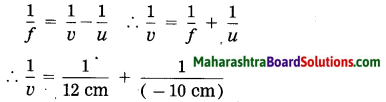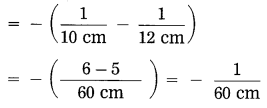∴ v = -60 cm
It is negative.
The image is formed at 60 cm from the lens and on the same side of the lens relative to the object. It is virtual, erect, and enlarged.

Problem 9.
An object of height 4 cm is placed in front of a concave lens of focal length 40 cm. If the object distance is 60 cm, find the position and height of the image.
Solution:
Data: f = -40 cm (concave lens),
u = -60 cm, h1 = 4 cm, v = ?, h2 = ?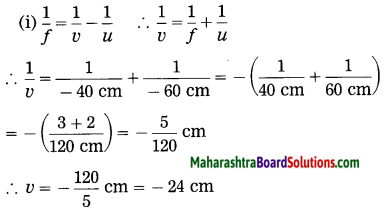The image Is formed at 24 cm from the lens.
It is on the same side as the object.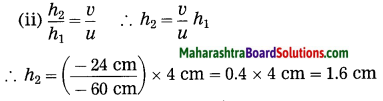The height of the image is 1.6 cm.

Problem 10.
What is the power of a convex lens having focal length 0.5 m?
Solution:
Data: Convex lens, f= 0.5 m, P = ?
P = 1𝑓=10.5m = 2D
The power of the lens = 2D.

Problem 11.
The power of a convex lens is 2.5 dioptres. Find its focal length.
(OR)
Calculate the focal length of a corrective lens having power +2.5 D.
Solution:
Data: Convex lens, P = +2.5 D, f = ?
P = 1𝑓
∴ 2.5 D = 1𝑓
∴ f = 12.5D = 0.4 cm = 40 cm
The focal length of the lens = 40 cm.

Problem 12.
Two convex lenses of focal length 20 cm each are kept in contact with each other. Find the power of their combination.
Solution:
Data: f1 = 20 cm = 0.2 m,
f2 =20 cm = 0.2 m, P (combination) = ?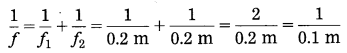∴ Focal length of the combination or the lenses, f = 0.1 m.
P = 1𝑓=10.1m = 10 D
The power of the combination of the lenses, P = 10 D.

Problem 13.
Two convex lenses of equal focal lengths are kept in contact with each other. If the power of their combination is 20 D, find the focal length of each convex lens.
Solution:
Data: Convex lens, P = 20 D, f1 = f2 = ?
The focal length (f) of the combination of the lenses is given by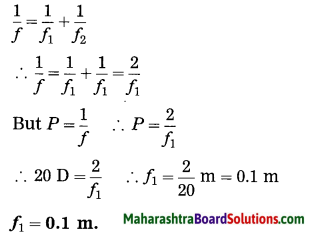This gives the focal length or each convex lens.

Problem 14.
If a convex lens of focal length 10 cm and a concave lens of focal length 50 cm are kept in contact with each other, (i) what will be the focal length of the combination? (ii) what wiil be the power of the combination? (iii) what will be the behaviour of the combination (behaviour as a convex lens/concave lens)?
Solution:
Data: f1 = +10 cm = +0.1 m (convex lens),
f2 = -50 cm = -0.5 m (concave lens),
f (combination) = ?, P (combination) = ?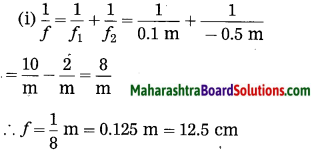The focal length or the combination of the lenses = 0.125 m = 12.5 cm.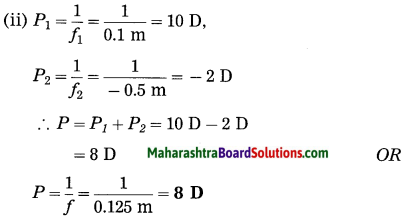The power of the combination of the lenses 8.D.
(iii) The focal length of the combination of the lenses is positive. This shows that the combination will behave as a convex lens.

Numerical problems for practice:

Problem 1.
Find the focal length of a convex lens which produces a real image at 60 cm from the lens when an object is placed at 40 cm in front of the lens.
24 cm

Problem 2.
Find the focal length of a convex lens which produces a virtual image at 10 cm from the lens when an object is placed at 5 cm from the lens.
10 cm

Problem 3.
A real image is obtained at 30 cm from a convex lens of focal length 7.5 cm. Find the distance of the object from the lens.
u = -10 cm

Problem 4.
An object is kept at 20 cm in front of a convex lens and its real image is formed at 60 cm from the lens. Find (1) the focal length of the lens (2) the height or the image if the height of the object is 6 cm.
(1) 15 cm
(2) h2 = -18 cm]

Problem 5.
An object is kept at 10 cm in front of a convex lens. Its image is formed on the screen at 15 cm from the lens. Calculate (1) the focal length of the lens (2) the magnification produced by the lens.
(1) 6 cm
(2)M = -1.5

Problem 6.
An object is kept at 60 cm in front of a convex lens of focal length 15 cm. Find the image distance and the nature of the image. Also find the magnification produced by the lens.
v = 20 cm. The image is real, inverted and smaller than the object. M = –13]

Problem 7.
An object of height 2 cm is kept at 30 cm from a convex lens. Its real image is formed at 60 cm from the lens. Find the focal length and power of the lens.
f = 20 cm, P = 5 D

Problem 8.
If the power of a lens is 4 dioptres, find its focal length.
25 cm

Problem 9.
Find the power of a convex lens of focal length 40 cm.
2.5 D

Problem 10.
Find the power of a convex lens of focal length 12.5 cm.
8 D

Problem 11.
If for a lens, f = – 20 cm, what is the power of the lens?
-5 D

Problem 12.
An object of height 4 cm is kept in front of a concave lens of focal length 20 cm. If the object distance is 30 cm, find the position and the height of the image.
v = -12 cm, h2 = 1.6 cm

Problem 13.
If two convex lenses of focal lengths 10 cm and 5 cm are kept in contact with each other, what is their combined focal length?
103 cm [approximately 3.33 cm]

Problem 14.
If a convex lens of focal length 20 cm and a concave lens of focal length 30 cm are kept in contact with each other, (i) What will be the focal length of the combination? (ii) What will be the power of the combination? (iii) What will be the behaviour of the combination?
(i) f = 60 cm
(ii) P = 53 D = 1.6667 D (approximately)
(iii) The combination will behave as a convex lens.

Problem 15.
A concave lens of focal length 12 cm and a convex lens of focal length 20 cm are kept in contact with each other, (i) Find the focal length of the combination, (ii) What will be the behaviour of the combination?
(i) f = -30 cm
(ii) The combination will behave as a concave lens.

## निष्कर्ष

आम्हाला आशा आहे की महाराष्ट्रावरील हा लेख तुम्हाला 'दहावी विज्ञान धडा लेन्स स्वाध्याय' मदत करेल. तुम्हाला काही प्रश्न असल्यास, त्यांना खाली टिप्पणी विभागात मोकळ्या मनाने पोस्ट करा. आम्ही लवकरात लवकर तुमच्याकडे परत येऊ.
Share: